### Deep Differentiable Logic Gate Networks & their (potential) relevance to ZKML

This paper (arXiv), authored by Petersen, Borgelt, Kuehne and Deussen, was accepted for NeurIPs 2022. It does one of my favourite things: it learns a discrete structure (in this case, a boolean circuit) via differentiable means. Logic gate networks came up in a recent ZKML discussion as a machine learning paradigm of interest: since boolean circuits are encodable as arithmetic circuits, proving inference runs of a logic gate network can be done without the loss of accuracy that is inherent in the quantization approach to making the more familiar neural nets amenable to ZK. In fact, the situation is more subtle than that: a logic gate network is in fact discretized before inference (just not by us), and there was necessarily a small loss in accuracy in that process. An important difference, however, is that logic gate networks have been designed with this discretization in mind, since this is exactly what needs to be done to make model inference fast on edge hardware. This post presents notes on a presentation on this topic and the questions that arose during the discussion.

## What’s a deep differentiable logic gate network?

A differentiable logic gate network consists of multiple layers of “neurons” with random wiring: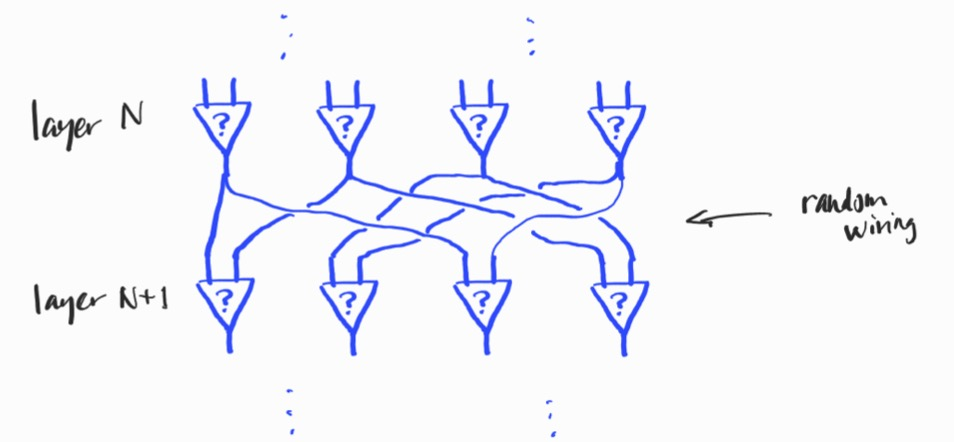Each neuron has precisely two inputs and is a superposition of the possible binary logic gates:where the probabilities (in purple) are learned from the data (using the softmax parameterization).

During training, the logic gates operate on values in the interval $[0,1]$ via arithmetic expressions e.g. $$\mathrm{OR}(x,y) = x + y – xy,$$and the output of a neuron is the expected value, over all logic gates, of their respective outputs, i.e. it’s a sum of the outputs of each gate, weighted by the probability of that gate (the purple values). Post-training, the network is discretized to yield a boolean circuit by replacing each neuron with its most likely logic gate.

## Novelty

The superposition of different architectural components (logic gates) via the softmax is, to the best of my knowledge, novel. Note that here, softmax is not an activation function. Moreover, it’s not similar to the use of softmax in attention, as the probabilities are independent of the current input.

## Points of interest

### Why all 16 possible gates?

There are 16 possible binary logic gates – just count the boolean functions of two variables. Even though a smaller variety of gates would suffice to express any boolean circuit, the authors allow all 16, and this at the expense of increasing the training time, since there are more outcomes in the softmax to parameterise. The authors talk about this in the paper themselves. However, very deep circuits are hard to learn in their regime, so this redundancy is purposeful here.

### Deep(er) logic gate networks are hard to learn

The authors explain this in terms of vanishing gradients. I think the problem is more fundamental, having nothing to do with the gradient, per se. An expectation is taken at every neuron/node in the circuit, and the layering of these successive expectations means that the signal at the output layer is very diluted. It is interesting to reflect that what we really would like to do is perform a superposition over all possible circuits of some size. This is of course infeasible, since the number of such circuits would be astronomical. So the authors perform superpositions of logic gates at each node, instead. Since deep logic gate networks are hard to learn (the maximum depth used by the authors is 6 layers), the authors go wide: their largest network has 1M nodes on each layer!

### The wiring is fixed

The wiring of the network is the pattern of connections from layer to layer. Familiar neural networks are often fully connected, so each neuron is connected to all neurons in the previous layer. For logic gate networks, this is not the case: each neuron receives precisely two inputs from the outputs of the previous layer. There are obviously a huge number of choices for how to wire two layers together. In the approach of the authors, for simplicity, the wiring is determined pseudo-randomly before training and fixed for all time.

The authors don’t count the connection information in the storage requirements for the model, since the connections are determined by the initial random seed. This isn’t wrong, but it’s worth remembering (as the authors themselves note) that any future approach that employs a more intelligent method of determining the wiring will need to store the connection information.

### Learning the wiring would be better

Learning the wiring would likely mean (in my estimation) that the such wide layers wouldn’t be necessary: a huge number of random connections would no longer be required to find the favourable information flows from inputs to outputs. Learning the wiring would be a non-trivial optimisation problem, however, more complicated than e.g. general sparsity constraints since the number of inputs must be exactly two (not just a arbitrary small number).

### Regression as well as classification?

The authors also undertake to demonstrate that logic gate networks, when appended with a learned affine transformation, are useful devices for regression. This is not without precedent, since e.g. sometimes random forests are used for regression. However, I think the core case of classification is compelling enough.

### The “normalisation temperature” $\tau$

Outputs are grouped into equally sized groups corresponding to the classes of the classification, and the score for each class is the sum of its outputs. These sums need normalising, since the resultant “score vector” needs to be a distribution: it is compared to the target distribution (which is one-hot on the target class) using cross entropy. There is only one correct way to do this, namely normalize by the size of the groups. Instead, the authors normalize by a hyperparameter $\tau$, for which they perform a grid search. The beneficial side effect of varying the value of $\tau$ is to amplify or diminish the strength of the back propagation signal, and as such, it ends up playing the role of a learning rate (the hyperparameter that the authors identify as a learning rate is in fact fixed).

### Surprisingly, no annealing!

It is common practice to use a “temperature” parameter in the softmax to progressively encourage the model to move towards making a one-hot decision – this is called annealing. Surprisingly, the authors choose not to do this, and perhaps they didn’t need to (see Discretization).

### Discretization

The authors claim that, post-training, the model has learned an unambiguous choice of logic gate for each node; they are consequently able to discretize the network of superpositions into a boolean circuit. This of course damages the accuracy of the model. The authors report however that this lose of accuracy is less that 0.1% for MNIST.

(I was skeptical about the claim that the choice of logic gate would be unambiguous post training, but can confirm that this is indeed the case: after training the smaller MNIST model, >96% of all neurons on all layers were essentially one-hot (i.e. one outcome had probability >85%).

## Relevance to ZKML

Logic gate networks belong to the broad family of approaches that are focussed on efficient inference for edge devices, which includes further model types that may be of interest for ZKML, such as binary neural nets, quantised weight networks and neural nets trained with sparsity constraints. The other feature that all such models have in common is that they sacrifice some accuracy for efficiency and are consequently never the latest and greatest in performance. However, logic gate networks in particular are still in the first stages of their development, and future directions for improvement are apparent and, in my opinion, feasible.

The most important future improvement will be lower redundancy. Logic gate networks (in their current state) are boolean circuits with a high degree of redundancy resulting from their learning process. Reducing this redundancy will be crucial for making such models useful beyong toy datasets. For example, about four million logic gates are required for a competitive logic gate network for MNIST. From the ZKML perspective, to prove an inference run of such a network, the output of each logic gate (a bit) needs to be encoded as a separate field element – they can’t be grouped together into a larger field element since bit-wise operations need to be verified. While this is not so onerous (depending on the proof system), this is still just a model for a toy dataset. What is needed is a method of learning with reduced redundancy, and this will hopefully be the result of future work.

### Elliptic curve pairings: a first example

Here is a nice simple example of bilinear pairing on an elliptic curve over a finite field. Different sorts of pairings exist – here we’ll construct the Weil pairing, and for simplicity we’ll do it in a direct way that avoids talking about divisors on algebraic curves.

Consider the elliptic curve over $\mathbb{F}_7$ defined by the equation $$y^2 = x^3 + 2.$$ There are nine solutions: $$\{ \mathcal{O},\ (0, \pm 3),\ (3, \pm 1),\ (5, \pm 1),\ (6, \pm 1)\ \}.$$ The identity element $\mathcal O$ is a point at infinity corresponding to the vertical axis (it is a point of the projective plane). All the other points are elements of the affine plane $\mathbb{F}_7 \times \mathbb{F}_7$. These nine points form a group which we’ll denote by $\mathbb{G}$.

We can check that for any $P \in \mathbb{G}$, we have $3 P = \mathcal O$. The most elementary way to check this is by direct calculation: we need to check that $P + P = -P$ for each point $P$. Recalling the diagrammatic rule for computing $P+P$, we need to draw the tangent to the curve at $P$, and then find the other point of intersection with the curve (it turns out this geometric interpretation of point doubling works over prime fields as well). So let’s calculate the slope $s$ of the tangent at each point $P=(x,y)$:
$$s = \frac{\frac{\partial}{\partial x} RHS}{\frac{\partial}{\partial y} LHS} = \frac{\frac{\partial}{\partial x} (x^3 + 2)}{\frac{\partial}{\partial y} y^2} = \frac{3x^2}{2y}.$$
This gives us the following diagram:

The dashed blue lines indicate the tangents to the curve at each point. Check for yourself that if you follow any of these tangents (remembering to wrap around to the opposite side when you get to an edge!) then you don’t hit any other points, i.e. the point of tangency $P$ is the only point of the elliptic curve on the tangent line, meaning that the “other” point of intersection must be $P$ itself (this means it’s an intersection of “higher order”). So $P + P$ is equal to the reflection of $P$ through the line $y=0$, i.e. $-P$, and so $3 P = \mathcal O$, as claimed.

In fact, it turns out that $\mathbb{G} \cong \mathbb{Z}_3 \times \mathbb{Z}_3$ as groups; you can construct such an isomorphism yourself by setting e.g. $g_1 = (0,3)$ and $g_2 = (3, 1)$ and taking these as the generators of the two copies of $\mathbb{Z}_3$ in the direct product. Note that this choice of isomorphism is not canonical (i.e. there is nothing to justify this particular choice) but that’s fine for our purposes. All we really need is that any $g \in \mathbb{G}$ has a unique expression $g = a_1 g_1 + a_2 g_2$ as a $\mathbb{Z}_3$-linear combination of our chosen generators $g_1, g_2$.

We are finally in a position to define a bilinear pairing. There are various types of pairings. Here, we’ll construct the Weil pairing. Let $\mu$ be an abelian group, written multiplicatively. We want a map $e: \mathbb{G} \times \mathbb{G} \to \mu$ that is bilinear, i.e. $$e(g + g’, h) = e(g, h) \cdot e(g’, h), \quad e(g, h + h’) = e(g, h) \cdot e(g, h’),$$ for all $g, g’, h, h’ \in \mathbb{G}$, and alternating, i.e. $$e(g, h) = e(h, g)^{-1} \quad \forall g, h \in \mathbb{G}.$$ Some further properties follow immediately. For example, using that $\mathcal{O} + \mathcal{O} = \mathcal{O}$, it follows from bilinearity that $$e(g, \mathcal{O}) = e(\mathcal{O}, h) = 1 \in \mu,$$ and from this last property and the fact that $3 P = \mathcal{O}$ for all $P \in \mathbb{G}$, it follows that $$e(g, h)^3 = 1 \quad \forall g, h \in \mathbb{G},$$ and so we might as well take $\mu = \mu_3$ to be the group of third roots of unity over $\mathbb{F}_7$. Note $\mathbb{F}_7$ in fact contains all its third roots of unity, since $2^3 \equiv 1$ modulo $7$.

So how many alternating, bilinear forms can be there be on $\mathbb{G}$? Up to a choice of third root of unity, just one! To see this, just use the bilinearity and alternation properties to check that
$$e(a g_1 + b g_2, c g_1 + d g_2) = e(g_1, g_2)^{ad – bc}, \quad \forall a,b,c,d \in \mathbb{Z}_3,$$
i.e. all such forms are given by some third root of unity $e(g_1, g_2)$ raised to the power of the determinant of the matrix of coefficients. And on the other hand, since we know that the determinant is an alternating multilinear function on matrix rows, we see that this definition really does give us an alternating bilinear form on $\mathbb{G}$. From uniqueness, we know that this pairing must in fact coincide (up to choice of root of unity) with the Weil pairing of our elliptic curve, since it too is alternating and bilinear.

What about the general case? The Weil pairing is defined on the “$r$-torsion” $E[r]$ of an elliptic curve, i.e. the subgroup of points $P$ such that $r P = \mathcal{O}$. In our example, $r=3$ and the $3$-torsion subgroup $E$ coincides with the entire group $\mathbb{G}$ (this is rarely true). On the other hand, the group isomorphism $E[r] \cong \mathbb{Z}_r \times \mathbb{Z}_r$ holds in general, with the caveat that you’ll likely need to take a non-trivial extension of a prime field as your base field. From there, the construction outlined above in terms of the determinant could be used. However, in order to prove useful things about the pairing, the divisor-theoretic construction is much more useful, since it allows us to call on the theory of algebraic curves (and in fact this is needed to even prove that $\mathbb{G}$ is a group!). It is also worth noting that in order to actually calculate a Weil pairing using the determinant construction, it is necessary to express an arbitrary $g \in E[r]$ as a linear combination of the chosen generators (and this problem would be hard even if there was just one generator: that’s the discrete logarithm problem!). So there are good reasons for the definition of the Weil pairing in terms of divisors, after all.

Addendum #1: as an alternative to the slope/tangent method above for visually checking that each point has order $3$, you can check instead that the Hessian determinant $X (Y^2 – Z^2)$ of the homogenisation of the equation defining the elliptic cruve vanishes at each point, i.e. show that each point is a point of inflection. See Silverman’s The Arithmetic of Elliptic Curves, exercise III.3.9.

Addendum #2: The example above has been chosen so that everything can be calculated by hand. To work with other examples, or to check your work, Magma is a great help. Here is some example code for the above calculations, which you can plug into the online calculator:

K := GF(7);  // the finite field with 7 elements

// The EC with equation y^2 = x^3 + 0*x + 2 over K
E := EllipticCurve([K | 0, 2]);
print E, "\n";

print "Number of points on the curve", #E, "\n";

// Creation of a point with given projective coordinates
P := E ! [6, -1, 1];

print "The order of each element:";
for P in Points(E) do
print "Element", P, "has order", Order(P);
end for;
print "\n";

print "Point doubling:";
for P in Points(E) do
print "2", P, "=", P+P;
end for;
print "\n";

print "Find a decomposition of E as a product of finite cyclic groups:";
AbelianGroup(E);
print "\n";

print "Calculate the Weil pairing:";
for P in Points(E) do
for Q in Points(E) do
P, "paired with", Q, "=", WeilPairing(P, Q, 3);
end for;
end for;

### A toy elliptic curve over a finite field

Here is a first example of an elliptic curve over a finite field where you can work everything out by hand.

Consider the elliptic curve defined by the equation
$$y^2 = (x-1)(x-2)(x-3)$$
over the field $\mathbb{F}_5$. Multiplying out the right hand side, we see that (over $\mathbb{F}_5$), the right hand side (“RHS”) is $x^3 – x^2 + x – 1$. So our equation is not in Weierstrauss form, but that’s fine. It’s still defines an elliptic curve. Note that we know immediately that it is non-singular, since the roots of the RHS are distinct.

It’s easy to find all the solutions $(x,y)$ to our equation by hand – just consider each possible value for $x \in \mathbb{F}_5$, calculate the RHS, and set $y = \pm \sqrt{RHS}$, if such $y$ exist. So we first need to know which values in $\mathbb{F}_5$ are squares:

$$\begin{array}{c|ccccc} z & 0 & 1 & 2 & 3 & 4 \\ z^2 & 0 & 1 & 4 & 4 & 1 \end{array}$$

Thus the points on our elliptic curve are
$$(0,2), (0,3), (1,0), (2,0), (3,0), (4,1), (4,4), \mathcal{O}.$$
The point $\mathcal{O}$ is the solution at infinity: this is the one extra solution $(0:0:1)$ that arises when the equation defining the elliptic curve is homogenized, and solutions in the projective plane are permitted. The other solutions live in the “affine” $(x,y)$ plane.

The nice thing about working over an unextended finite field like $\mathbb{F}_5$ is that it is still “1-dimensional”, so the affine solutions $(x,y)$ can be depicted on a 2-dimensional diagram like the following:

Fortunately, the familiar geometric description of the group operation on elliptic curves in terms of line intersections still works (why?). That is, any two points can be added by drawing a line through them, finding the third point of intersection, and reflecting through the line $y=0$, and the point $\mathcal{O}$ corresponds to the vertical direction and is the identity element of the group.

For example, it is immediate from this rule that $A+B=C$. Remembering that lines wrap-around our diagram (which is actually a torus), what do you think $C+D$ is equal to? (Hint: it’s the next letter of the alphabet).

As in the case over $\mathbb{R}$:

• If a vertical line passes through two distinct affine points such as $(0,2)$ and $(0,3)$, then (since it also intersects with $\mathcal{O}$ in the projective plane) these points are inverses of one another w.r.t. the group operation. (We’ve labelled $-D, -E$ to reflect this.)
• If a vertical line hits a single affine point (e.g. the line $x=1$) then this point is its own inverse.

Thus $A, B, C$ are all group elements of order 2.

Amusingly, the geometric rule for point doubling using tangents still works, as well. The slope of the tangent at a point $(x,y)$ on our elliptic curve can be calculated in the usual way.
$$s = \frac{\frac{\partial}{\partial x} RHS}{\frac{\partial}{\partial y} LHS} = \frac{3x^2 – 2x + 1}{2y}.$$
These slopes are depicted on our diagram with dashed blue lines. Following these tangents, you can immediately verify that
$$\pm E + \pm E = B, \qquad \pm D + \pm D = B,$$
and so $\pm D, \pm E$ have order $4$ as group elements.

The orders of our group elements are enough to conclude that our group (call it $\mathbb{G}$) is isomorphic to $\mathbb{Z}_2 \times \mathbb{Z}_4$. Indeed, since $A+B=C$ and $A+D=E$ (to check, just follow the lines!) we have that
$$\begin{array}{ccc} \mathcal{O} & \mapsto & (0,0) \\ A & \mapsto & (1,0) \\ B & \mapsto & (0,2) \\ C & \mapsto & (1,2) \\ \pm D & \mapsto & (0,\pm 1) \\ \pm E & \mapsto & (1,\pm 3)\\ \end{array}$$
is an isomorphism of groups $\mathbb{G} \rightarrow \mathbb{Z}_2 \times \mathbb{Z}_4.$

### Siegelmann & Sontag’s “On the Computational Power of Neural Nets”

Here are the slides from a talk I gave the Sydney machine learning meetup on Siegelmann and Sontag’s paper from 1995 “On the Computational Power of Neural Nets”, showing that recurrent neural networks are Turing complete. It is a fantastic paper, though it is a lot to present in a single talk. I spent some time illustrating what the notion of a 2-stack machine, before focussing on the very clever Cantor set encoding of the authors.

There are also the following notes from a talk I gave at our Berlin ML seminar.

### Graph embeddings in Hyperbolic Space

I gave a talk last night at the Berlin machine learning meetup on learning graph embeddings in hyperbolic space, featuring the recent NIPS 2017 paper of Nickel & Kiela. Covered are:

• An illustration of why the Euclidean plane is not a good place to embed trees (since circle circumference grows only linearly in the radius);
• Extending this same argument to higher dimensional Euclidean space;
• An introduction to the hyperbolic plane and the Poincaré disc model;
• A discussion of Rik Sarkar’s result that trees embed with arbitrarily small error in the hyperbolic plane;
• A demonstration that, in the hyperbolic plane, circle circumference is exponential in the radius (better written here);
• A review of the results of Nickel & Kiela on the (transitive closure of the) WordNet hypernymy graph;
• Some thoughts on the gradient optimisation (perhaps better written here).

And here are the slides!

### Gradient optimisation on the Poincaré disc

Nickel & Kiela had a great paper on embedding graphs in hyperbolic space at NIPS 2017. They work with the Poincaré ball model of hyperbolic space. This is just the interior of the unit ball, equipped with an appropriate Riemannian metric. This metric is conformal, meaning that the inner product on the tangent spaces on the Poincare ball differ from that of the (Euclidean) ambient space by only a scalar factor. This means that the hyperbolic gradient $\nabla_u$ at a point $u$ can be obtained from the Euclidean gradient $\nabla^E_u$ at that same point just by rescaling. That is, you pretend for a moment that your objective function is defined in Euclidean space, calculate the gradient as usual, and just rescale. This scaling factor depends on the Euclidean distance $r$ of $u$ from the origin, as depicted below: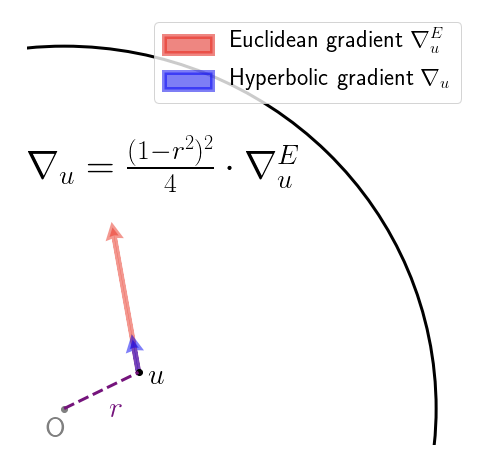So far, so good. What the authors then do is simply add the (rescaled) gradient to obtain the new value of the parameter vector, which is fine, if you only take a small step, and so long as you don’t accidentally step over the boundary of the Poincaré disc! A friend described this as the Buzz Lightyear update (“to infinity, and beyond!”). While adding the gradient vector seems to work fine in practice, it does seem rather brutal. The root of the “problem” (if we agree to call it one) is that we aren’t following the geodesics of the manifold – to perform an update, we should really been applying the exponential map at that current point to the gradient vector. Geodesics on the Poincaré disc look like this: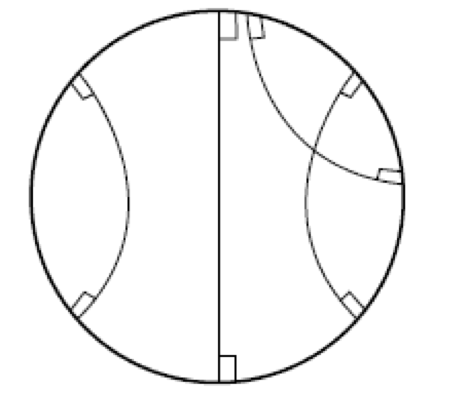that is, they are sections of circles that intersect the boundary of the Poincaré disc at right angles, or diameters (the latter being a limiting case of the former). With that in mind, here’s a picture showing how the Buzz Lightyear update on the Poincaré disc could be sub-optimal: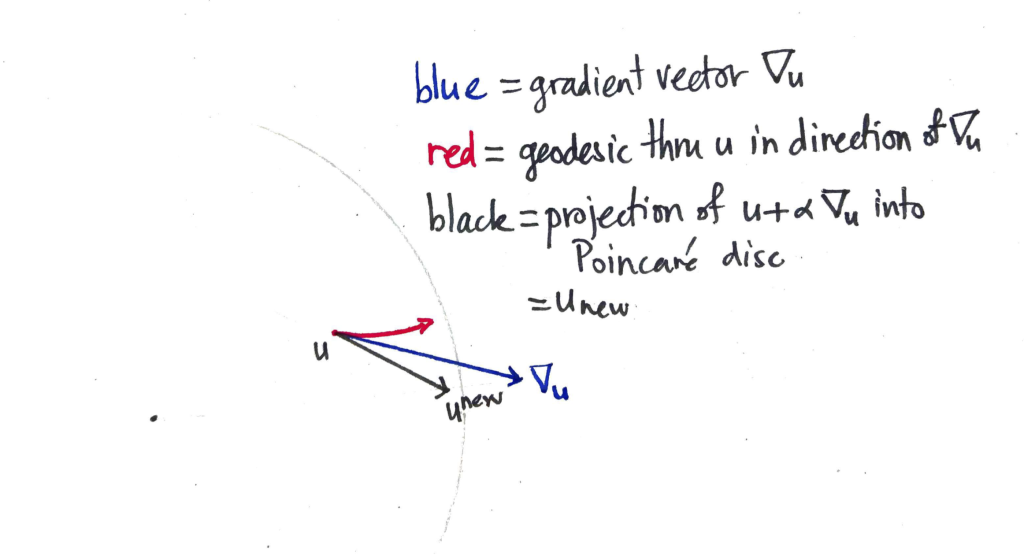The blue vector $\nabla_u$ is the hyperbolic gradient vector that is added to $u$, taking us out of the Poincaré disc. The resulting vector is then pulled back (along the ray with the faintly-marked origin) until it is within the disc by some small margin, resulting in the new value of the parameter vector $u^\textrm{new}$. On the other hand, if you followed the geodesic from $u$ to which the gradient vector is tangent, you’d end up at the end of the red curve. Which is quite some distance away.

### Circle circumference in the hyperbolic plane is exponential in the radius: proof by computer game

I recently needed to demonstrate this fact to an audience that I could not assume would be familiar with Riemannian geometry, and it took some time to find a way to do it! You can use the HyperRogue game, which takes place on a tiling of the Poincaré disc. The avatar moves across the Poincaré disc (slaying monsters and collecting treasure) by stepping from tile to tile. It is a pretty impressive piece of software, and you can play it on various devices for small change. Below are four scenes from the game. This image was taken from the interesting paper on HyperRogue by Kopczyński et al., cited at bottom.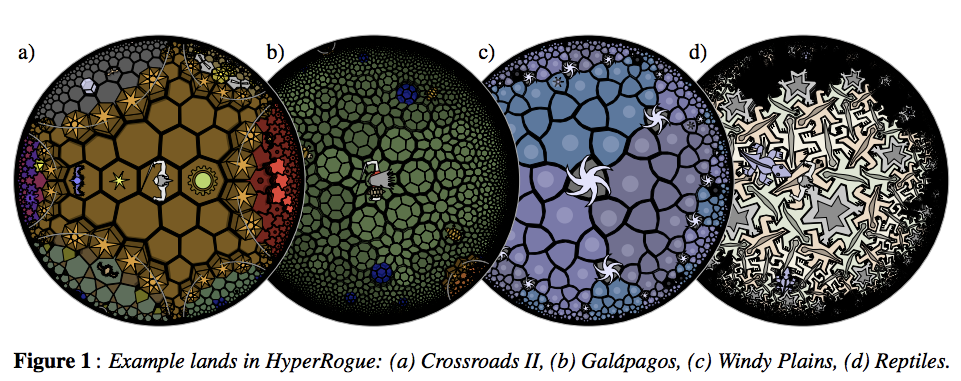As a proxy for proving that the growth rate was exponential, just count the number of tiles that were accessible (starting from the centre tile) in N steps and no less. This gives the sequence 1, 14, 28, 49, 84, 147, 252, 434, 749, … . Plotting this values gives evidence enough.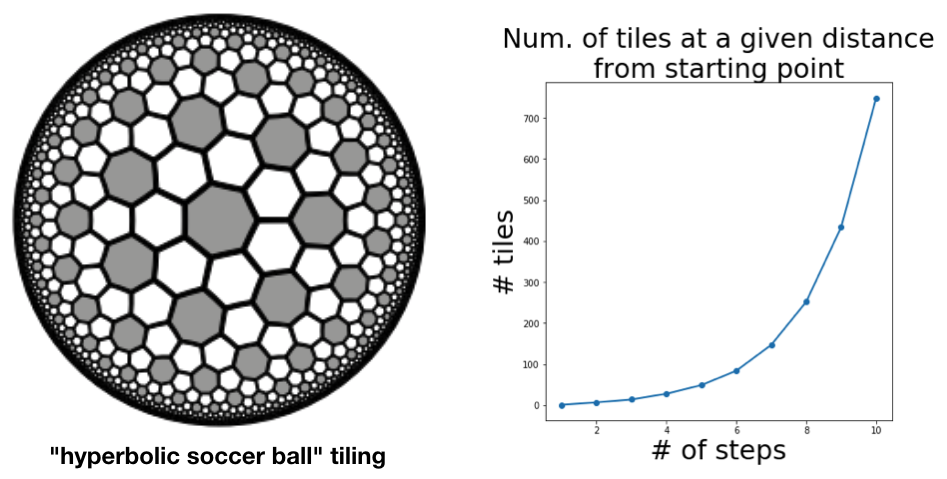The authors of the paper do a more thorough job, obtaining a recurrence relation for the sequence and using this to derive the base of the exponential growth (which comes out at about $\sqrt{3}$.

 Kopczyński, Eryk; Celińska, Dorota; Čtrnáct, Marek. “HyperRogue: playing with hyperbolic geometry”. Proceedings of Bridges 2017: Mathematics, Music, Art, Architecture, Culture (2017).

### Hierarchical Softmax

[These are the notes from a talk I gave at the seminar]

Hierarchical softmax is an alternative to the softmax in which the probability of any one outcome depends on a number of model parameters that is only logarithmic in the total number of outcomes. In “vanilla” softmax, on the other hand, the number of such parameters is linear in the number of total number of outcomes. In a case where there are many outcomes (e.g. in language modelling) this can be a huge difference. The consequence is that models using hierarchical softmax are significantly faster to train with stochastic gradient descent, since only the parameters upon which the current training example depend need to be updated, and less updates means we can move on to the next training example sooner. At evaluation time, hierarchical softmax models allow faster calculation of individual outcomes, again because they depend on less parameters (and because the calculation using the parameters is just as straightforward as in the softmax case). So hierarchical softmax is very interesting from a computational point-of-view. By explaining it here, I hope to convince you that it is also interesting conceptually. To keep things concrete, I’ll illustrate using the CBOW learning task from word2vec (and fasttext, and others).

The CBOW learning task is to predict a word $w_0$ by the words on either side of it (its “context” $C$).We are interested then in the conditional distribution $P(w|C)$, where $w$ ranges over some fixed vocabulary $W$.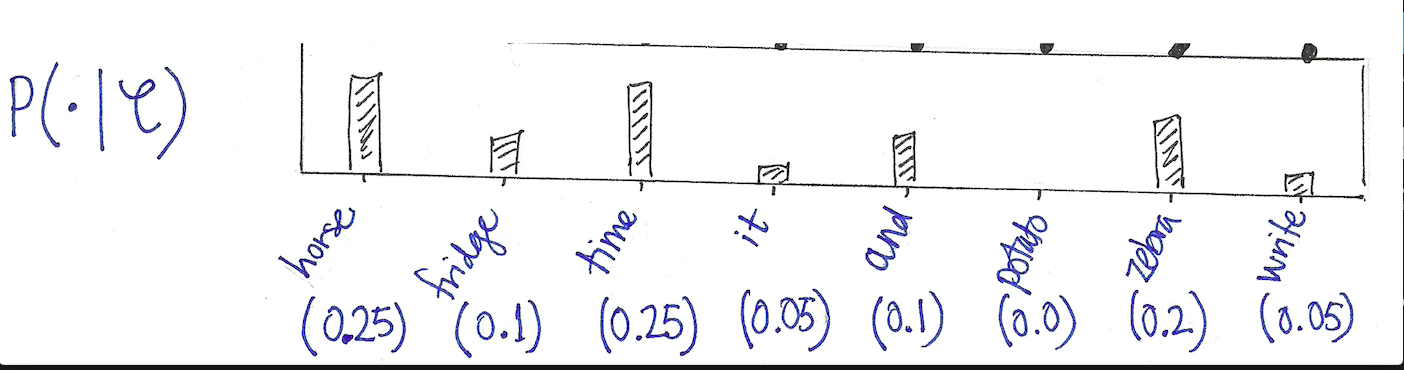This is very similar to language modelling, where the task is to predict the next word by the words that precede it.

## CBOW with softmax

One approach is to model the conditional distribution $P(w|C)$ with the softmax. In this setup, we have:

$$\hat{y} := P(w|C) = \text{exp}({\beta_w}^T \alpha_C) / Z_C,$$

where $Z_C = \sum_{w’ \in W} {\text{exp}({\beta_{w’}}^T \alpha_C)}$ is a normalisation constant, $\alpha_C$ is the hidden layer representation of the context $C$, and $\beta_w$ is the second-layer word vector for the word $w$. Pictorially: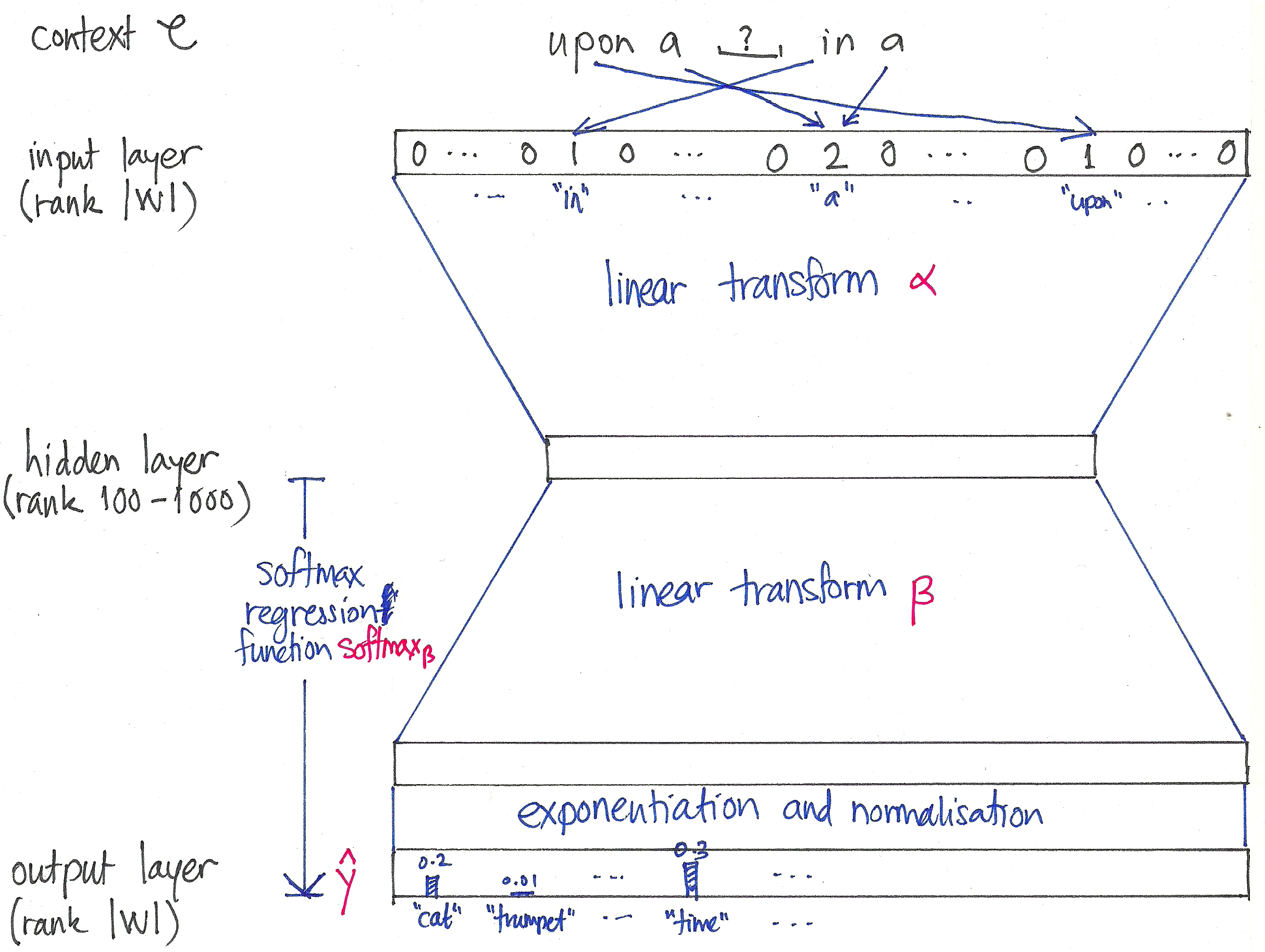The parameters of this model are the entries of the matrices $\alpha$ and $\beta$.

### Cross-entropy

For a single training example $(w_0, C)$, the model parameters are updated to reduce the cross-entropy $H$ between the distribution $\hat{y}$ produced by the model and the distribution $y$ representing the ground truth: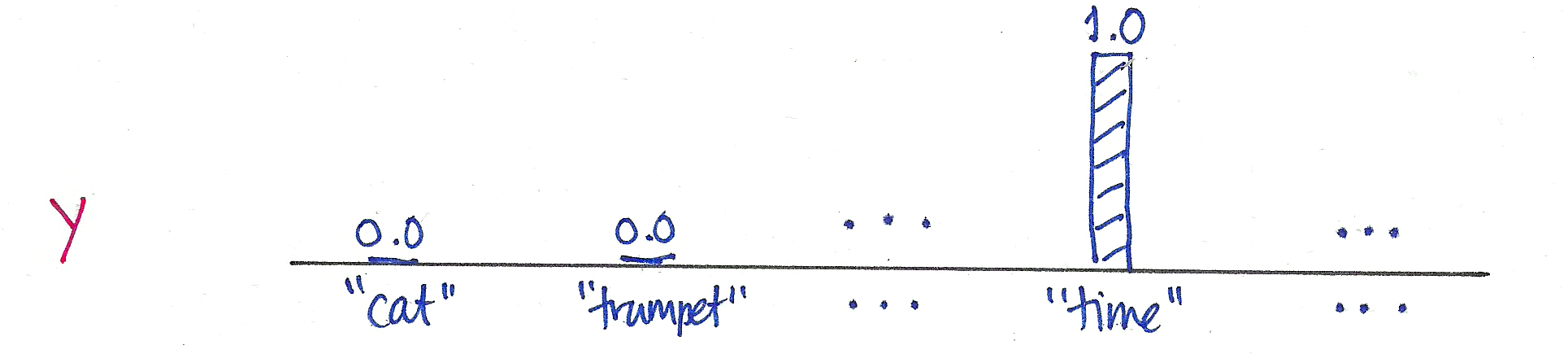Because $y$ is one-hot at $w_0$ (in this case, the word “time”), the cross-entropy reduces to a single log probability:
$$H(y, \hat{y}) := – \sum_{w \in W} {y_w \log \hat{y}_w = – \log P(w_0|C).$$
Note that this expression doesn’t depend on whether $\hat{y}$ is modelled using the softmax or not.

### Optimisation of softmax

The above expression for the cross entropy is very simple. However, in the case of the softmax, it depends on a huge number of model parameters. It does not depend on many entries of the matrix $\alpha$ (only on those that correspond to the few words in the context $C$), but via the normalisation $Z_C$ it depends on every entry of the matrix $\beta$. The number of these parameters is proportional to $|W|$, the number of vocabulary words, which can be huge. If we optimise using the softmax, all of these parameters need to be updated at every step.

## Hierarchical softmax

Hierarchical softmax provides an alternative model for the conditional distributions $P(\cdot|C)$ such that the number of parameters upon which a single outcome $P(w|C)$ depends is only proportional to the logarithm of $|W|$. To see how it works, let’s keep working with our example. We begin by choosing a binary tree whose leaf nodes can be made to correspond to the words in the vocabulary: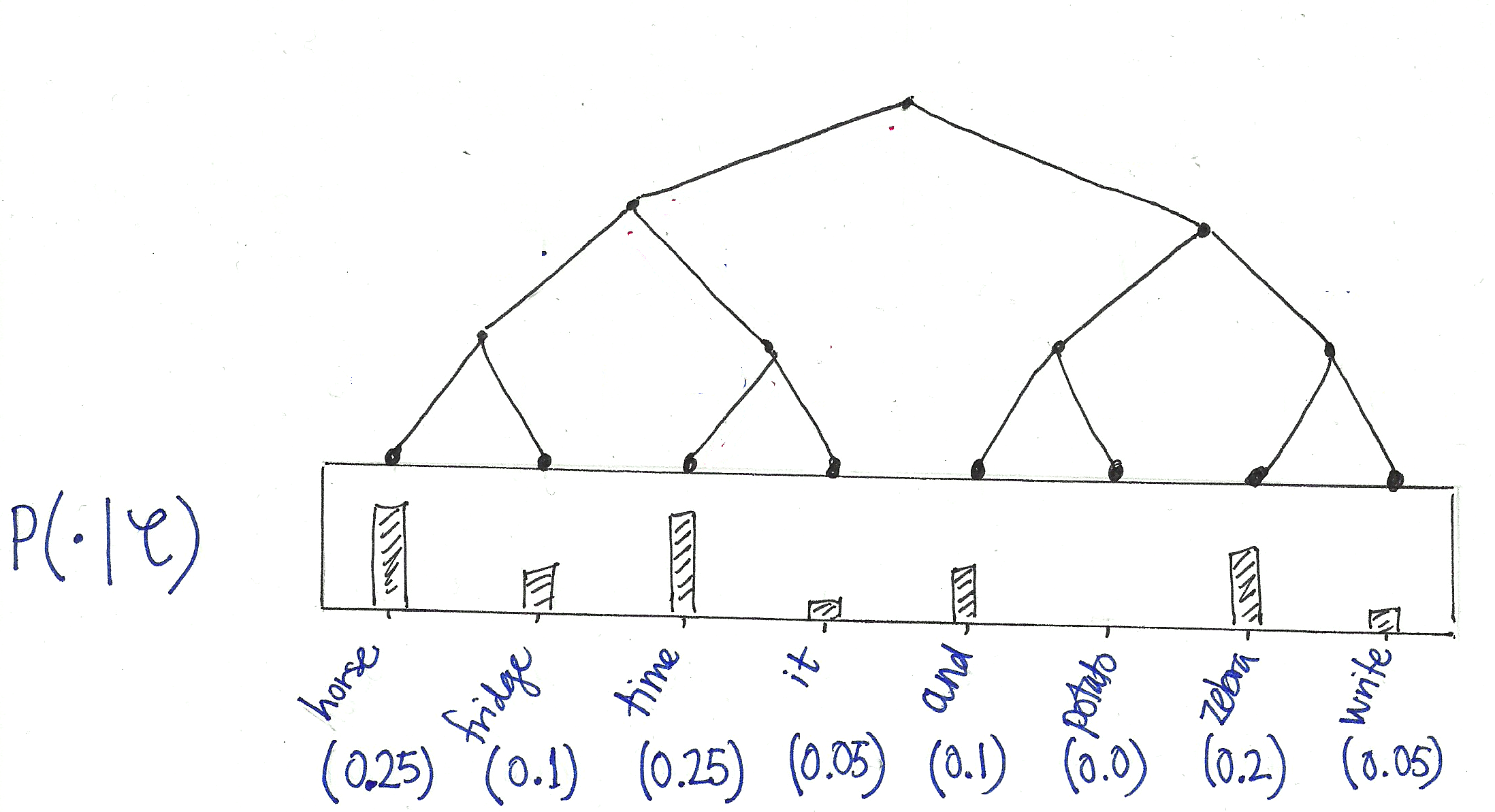Now view this tree as a decision process, or a random walk, that begins at the root of the tree and descents towards the leaf nodes at each step. It turns out that the probability of each outcome in the original distribution uniquely determines the transition probabilities of this random walk. At every internal node of the tree, the transition probabilities to the children are given by the proportions of total probability mass in the subtree of its left- vs its right- child: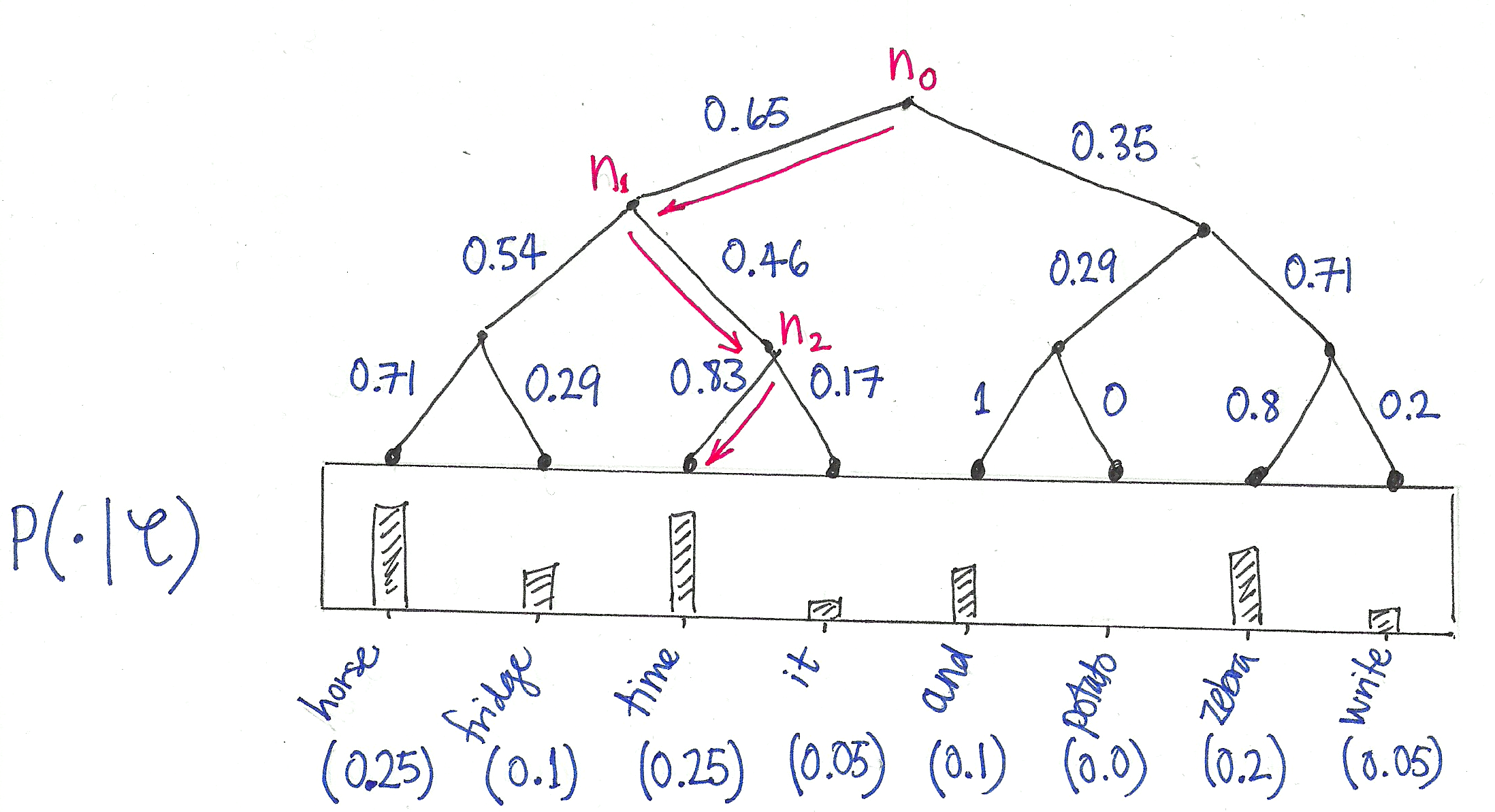This decision tree now allows us to view each outcome (i.e. word in the vocabulary) as the result of a sequence of binary decisions. For example:
$$P(\texttt{“time”}|C) = P_{n_0}(\text{right}|C) P_{n_1}(\text{left}|C) P_{n_2}(\text{right}|C),$$
where $P_{n}(\text{right}|C)$ is the probability of choosing the right child when transitioning from node $n$. There are only two outcomes, of course, so:
$$P_{n}(\text{right}|C) = 1 – P_{n}(\text{left}|C).$$
These distributions are then modelled using the logistic sigmoid $\sigma$:
$$P_{n}(\text{left}|C) = \sigma({\gamma_n}^T \alpha_C),$$
where for each internal node $n$ of the tree, $\gamma_n$ is a coefficient vector – these are new model parameters that replace the $\beta_w$ of the softmax. The wonderful thing about this new parameterisation is that the probability of a single outcome $P(w|C)$ only depends upon the $\gamma_n$ of the internal nodes $n$ that lie on the path from the root to the leaf labelling $w$. Thus, in the case of a balanced tree, the number of parameters is only logarithmic in the size $|W|$ of the vocabulary!

## Which tree?

### J. Goodman (2001)

Goodman (2001) uses 2- and 3-level trees to speed up the training of a conditional maximum entropy model which seems to resemble a softmax model without a hidden layer (I don’t understand the optimisation method, however, which is called generalised iterative scaling). In any case, the internal nodes of the tree represent “word classes” which are derived in a data driven way (which is apparently elaborated in the reference  of the same author, which is behind a paywall).

### F. Morin & Y. Bengio (2005)

Morin and Bengio (2005) build a tree by beginning with the “is-a” relationships for WordNet. They make it a graph of words (instead of word-senses), by employing a heuristicFelix, and make it acyclic by hand). Finally, to make the tree binary, the authors repeatedly cluster the child nodes using columns of a tf-idf matrix.

### A. Mnih & G. Hinton (2009)

Mnih & Hinto (2009) use a boot-strapping method to construct binary trees. Firstly they train their language model using a random tree, and afterwards calculate the average context vector for every word in the vocabulary. They then recursively partition these context vectors, each time fitting a Gaussian mixture model with 2 spherical components. After fitting the GMM, the words are associated to the components, and this defines to which subtree (left or right) a word belongs. This is done in a few different ways. The simplest is to associate the word to the component that gives the word vector the highest probability (“ADAPTIVE”); another is splitting the words between the two components, so that the resulting tree is balanced (“BALANCED”). They consider also a version of “adaptive” in which words that were in a middle band between the two components are placed in both subtrees (“ADAPTIVE(e)”), which results not in a tree, but a directed acyclic graph. All these alternatives they compare to trees with random associations between leaves and words, measuring the performance of the resulting language models using the perplexity. As might be expected, their semantically constructed trees outperform the random tree. Remarkably, some of the DAG models perform better than the softmax!

### Mikolov et al. (2013)

The approaches above all use trees that are semantically informed. Mikolov et al, in their 2013 word2vec papers, choose to use a Huffman tree. This minimises the expected path length from root to leaf, thereby minimising the expected number of parameter updates per training task. Here is an example of the Huffman tree constructed from a word frequency distribution: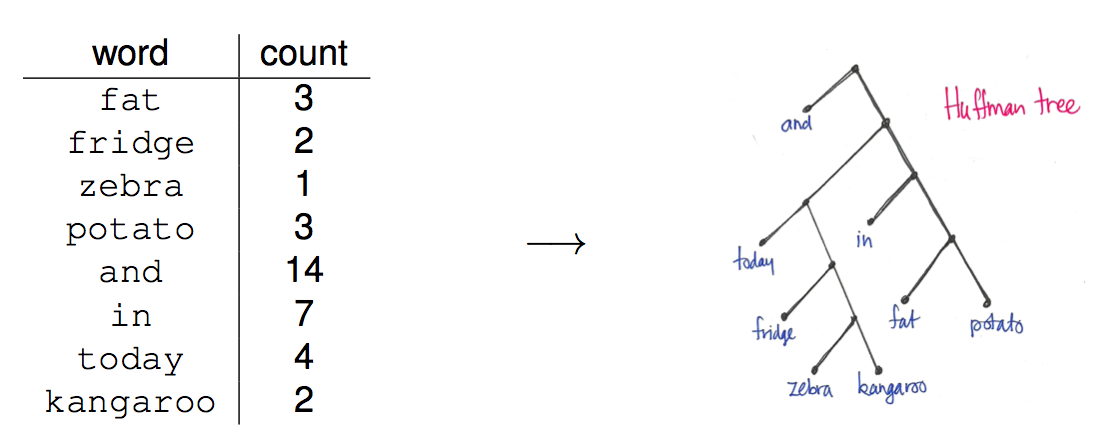What is interesting about this approach is that the tree is random from a semantic point of view.

### Minsky & Papert’s “Perceptrons”

In their book “Perceptrons” (1969), Minsky and Papert demonstrate that a simplified version of Rosenblatt’s perceptron can not perform certain natural binary classification tasks, unless it uses an unmanageably large number of input predicates. It is easy to show that with sufficiently many input predicates, a perceptron (even on this type) can perform any classification with perfect accuracy (see page 3 of the notes below). The contribution of Minsky and Papert is to show that meaningful restrictions on the type of input predicates hamper the expressive ability of the perceptron to such a degree that it is unable to e.g. distinguish connected from disconnected figures, or classify according to whether the number of active pixels is odd or even. The former has a simple picture proof, whereas the crucial ingredient for the latter is the action of a permutation group on the retina (i.e. the input array) of the perceptron.

## Talk

Here are my notes from a recent talk I gave on the book at the Berlin machine learning seminar:

## Style

The book is an engaging and instructive read – not only is it peppered with the author’s opinions and ideas, but it includes also enlightening comments on how the presented ideas originated, and why other ideas that occurred to the authors didn’t work out. The book still bears the marks of it’s making, so to speak.

## Controversy

The publication of the first edition in 1969 is popularly credited with bringing research on perceptrons and connectionism in general to a grinding halt. The book is held to be unjust, moreover. The “perceptrons”, which Minsky and Papert prove to be so limited in expressive power, were in fact only a very simplified version of what practitioners then regarded as a perceptron. A typical perceptron (unlike those of Minsky and Papert) might include more layers, feedback loops, or even be coupled with another perceptron. All these variations are described in Rosenblatt’s book “Principles of Neurodynamics” (1962). This is put very well (and colourfully!) by Block in his review of the book (1970):

Thus, although the authors state (p. 4, lines 12-14) “we have agreed to use the name ‘perceptron’ in recognition of the pioneer work of Frank Rosenblatt.”, they study a severely limited class of machines from a viewpoint quite alien to Rosenblatt’s. As a result, the title of the book, although generous in intent, is seriously misleading to the naive reader who wants to find out something about the general class of Perceptrons.

In summary then, Minsky and Papert use the word perceptron to denote a restricted subset of the general class of Perceptrons. They show that these simple machines are limited in their capabilities. This approach is reminiscent of the möhel who throws the baby into the furnace, hands the father the foreskin and says, “Here it is; but it will never amount to much.”

Despite these serious criticisms, it should be noted that Block (himself a trained mathematician) was full of praise for the “mathematical virtuosity” exhibited by Minsky and Papert in their book.

As to whether the book alone stopped research into perceptrons is hard to judge, particularly given it’s impact is confounded by the tragic death of Rosenblatt (at age 41) only two years later.

### Re-parameterising for non-negativity yields multiplicative updates

Suppose you have a model that depends on real-valued parameters, and that you would like to constrain these parameters to be non-negative. For simplicity, suppose the model has a single parameter $a \in \mathbb R$. Let $E$ denote the error function. To constrain $a$ to be non-negative, parameterise $a$ as the square of a real-valued parameter $\alpha \in \mathbb R$:

$$a = \alpha^2, \quad \alpha \in \mathbb R.$$

We can now minimise $E$ by choosing $\alpha$ without constraints, e.g. by using gradient descent. Let $\lambda > 0$ be the learning rate. We have

\begin{eqnarray*}
\alpha^{\text{new}} &=& \alpha – \lambda \frac{\partial E}{\partial \alpha} \\
&=& \alpha – \lambda \textstyle{\frac{\partial E}{\partial a} \frac{\partial a}{\partial \alpha}} \\
&=& \alpha – \lambda 2 \alpha \textstyle \frac{\partial E}{\partial a} \\
&=& \alpha \cdot (1 – 2 \lambda \textstyle \frac{\partial E}{\partial a})
\end{eqnarray*}

by the chain rule. Thus

\begin{eqnarray*}
a^{\text{new}} &=& (\alpha^{\text{new}})^2 \\
&=& \alpha^2 (1 – 2 \lambda \textstyle\frac{\partial E}{\partial a})^2 \\
&=& a \cdot (1 – 2 \lambda \textstyle\frac{\partial E}{\partial a})^2.
\end{eqnarray*}

Thus we’ve obtained a multiplicative update rule for $a$ that is in terms of $a$, only. In particular, we don’t need $\alpha$ anymore!

### Factorisation of stochastic matrices

Here we derive updates rules for the approximation of a row stochastic matrix by the product of two lower-rank row stochastic matrices using gradient descent. Such a factorisation corresponds to a decomposition

$$p(n|m) = \sum_k p(n|k) \cdot p(k|m)$$

Both the sum of squares and row-wise cross-entropy functions are considered.

### Heider and Simmel misinterpreted

I learnt of the 1944 experiment of Heider and Simmel in the Machine Intelligence workshop at NIPS 2016. The experiment involved showing subjects the video below, and asking them to describe what they saw. If you’ve watched the video, you’ll not be surprised to learn that most of the subjects anthropomorphised the geometric objects (i.e. they described them as humans, or their actions and intentions in human terms). In the talk at the workshop, this was offered as evidence that humans have a tendency to anthropomorphise, the implication then being that it is not hard to trick users into believing that e.g. a chat bot is a real person. While the implication might indeed be true, I don’t think the experiment of Heider and Simmel shows this at all. In my view, the reason that viewers anthropomorphise the shapes in the video is because they know that the video is made by human beings, and as such is created with intention. When you watch this video, you are participating in an act of communication, and the natural question to ask is: “what are the creators trying to communicate to me?”. For contrast, imagine that you are sitting in the bath and you have a few triangular shapes that float. If you float the shapes on the water and watch them for a while as they randomly (without expression of intent!) bob about, are you likely to anthropomorphise them? I’d say you are much less likely to do so.

### Wine dataset demonstrates importance of feature scaling

The UCI hosts a dataset of wine measurements that is fantastic for demonstrating the importance of feature scaling in unsupervised learning . There are a bunch of real-valued measurements (of e.g. chemical composition) for three varieties of wine: Barolo, Grignolino and Barbera. I am using this dataset to introduce feature scaling my course on DataCamp.

The wine measurements have very different scales, and performing a k-means clustering (with 3 clusters) on the unscaled measurements yields clusters that don’t correspond to the varieties:

varieties  Barbera  Barolo  Grignolino
labels
0               29      13          20
1                0      46           1
2               19       0          50


However, if the measurements are first standardised, the clusters correspond almost perfectly to the three wine varieties:

varieties  Barbera  Barolo  Grignolino
labels
0                0      59           3
1               48       0           3
2                0       0          65


Of course, k-means clustering is not meant to be a classifier! But when the clusters do correspond so well to the classes, then it is apparent that the scaling is pretty good.

### Which wine varieties?

I had to search to find the names of the wine varieties. According to page 9 of “Chemometrics with R” (Ron Wehrens), the three varieties are: Barolo (58 samples), Grignolino (71 samples) and Barbera (48 samples). I was unable to follow Wehren’s citation (it’s his ) on Google books.

### Original source

According to the UCI page, this dataset originated from the paper V-PARVUS. An Extendible Pachage of programs for esplorative data analysis, classification and regression analysis by Forina, M., Lanteri, S. Armanino, C., Casolino, C., Casale, M., Oliveri, P.

### Olive oil dataset

There is a dataset of olive oil measurements associated to the same paper. I haven’t tried using it, but am sure I’ll use it in an example one day.

### Eurovision song contest dataset

I’m teaching hierarchical clustering in my course on DataCamp, and I needed an interesting dataset. Importantly, I wanted the dataset to have labelled instances, so that the dendrogram would be easily interpretable, but also not too many instances, so they all fit on the dendrogram. Fortunately for me, the Eurovision song contest has been publishing the voting results (which is great!) and these are perfect. Both the voting results from the judges, and those from the public give great results. The only thing you need to adjust for is that countries are not allowed to vote for themselves in Eurovision, and this gives you some missing values in the data. I filled these with the maximum score of 12, since it is reasonable to assume that countries would vote selfishly if they were allowed to. Below is the dendrogram of a hierarchical (agglomerative) clustering using complete linkage.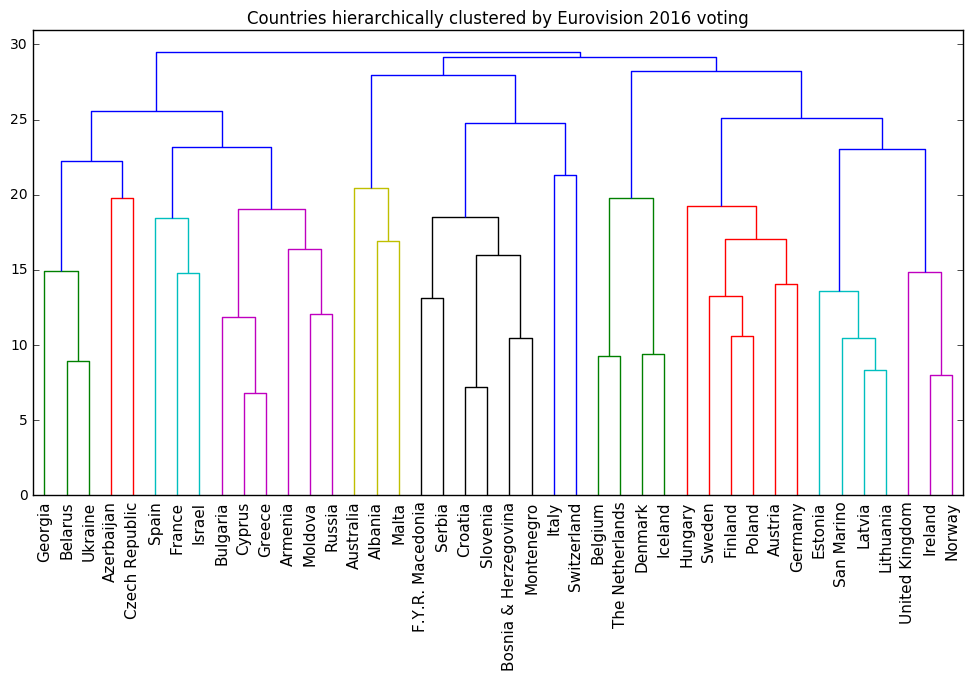### A better version

It occurs to me now that I should have normalised the rows after filling in the missing values. This does indeed improve the hierarchical clustering further.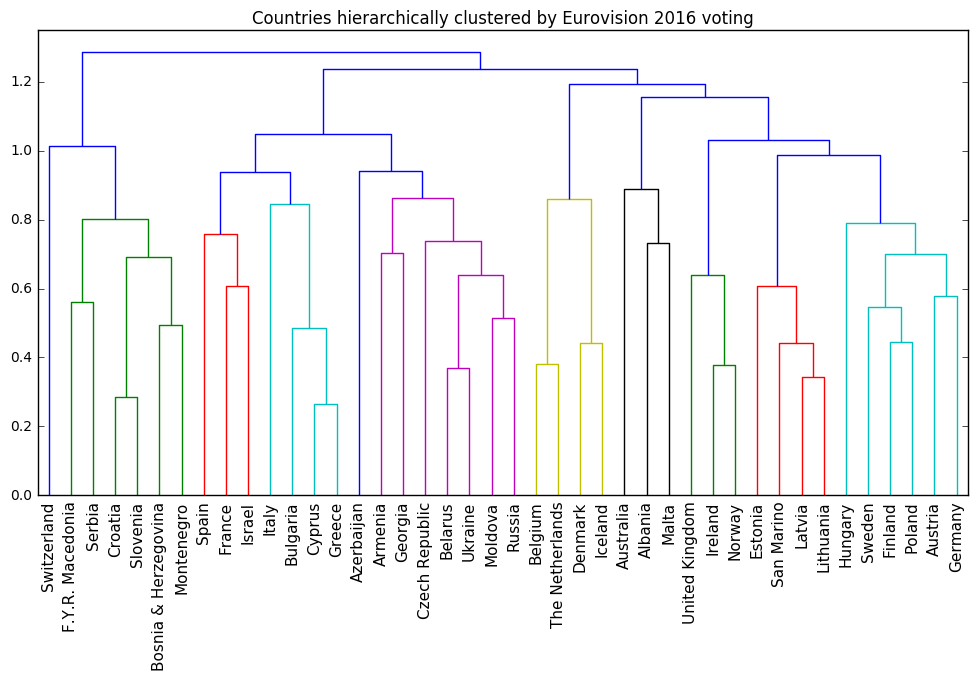### An LCD digit dataset for illustrating the “parts-based” representation of NMF

Non-negative matrix factorisation (NMF) learns to reconstruct samples as a superposition of their constituent parts. In the paper of Lee and Seung (1999) that popularised NMF, this is called a “parts-based” representation. This is illustrated in that paper by applying NMF to encodings of images of faces, where NMF seems to decompose the faces into a collage of eigen-eyebrows and eigen-noses. Visual demonstrations are fantastic for conveying ideas, but in this particular instance, the clarity is compromised by the inherent noisiness of real-world facial images. The images are drawn, moreover, from the CBCL dataset, which has a non-commercial license. In order to get around this problem, and to have an even clearer visual demonstration of the “parts-based” decomposition provided by NMF for my course at DataCamp, I created a synthetic image dataset, where each image is of a single digit of a LCD display, as on a clock radio. The parts learned by NMF are then the individual “cells” of the LCD display.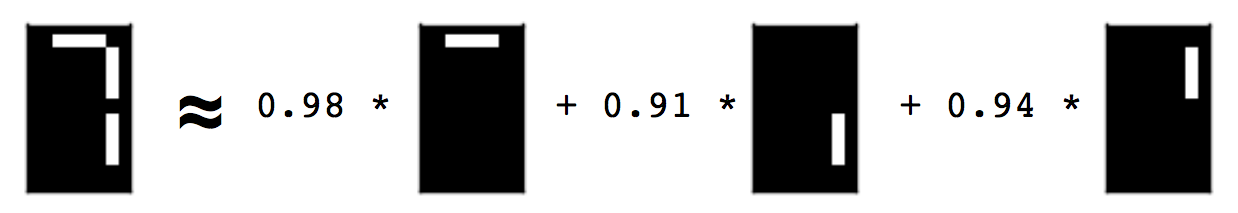You can construct this dataset yourself, using the code below. The collection of images is encoded as a 2d array of non-negative values. Each row corresponds to an image, and each column corresponds to a pixel. The non-negative entries represent the whiteness of the pixel, encoded here as a value between 0 and 1.

### Alternatives

• The standard bars provide a similar (but more apparently synthetic) image dataset for learning the parts of images. See, for example, the references given in Spratling (1996).
• Another great visual dataset could be built from black-and-white images of the 52 playing cards in a deck. NMF would then learn the ranks (i.e. ace, 2, 3, …, ) and the suits (i.e. spades, hearts, …) as parts, and reconstruct playing cards from these. I haven’t done this.
• Yet another great example dataset could be constructed using images of a piano keyboard, or perhaps just an octave range, colouring the keys according to how often they are pressed during a song. NMF should then be able to learn the chords as parts. The midi files to construct this dataset could be obtained from the Mutopia project, for example. I haven’t done this either.

### Don’t interpret linear hidden units, they don’t exist.

Having trained a model, it is natural to want to understand how it works. An intuitively appealing approach is to consider data samples that maximise the activation of a hidden unit, and to take the common input features of these samples as an indication of what that unit has learned to recognise. However, as we’ll see below, it is a misconception to speak of hidden units if:

• there is no non-linearity on the hidden layer;
• the weights connecting the layers are unconstrained; and
• the model is trained using (stochastic) gradient descent or similar.

In such a scenario, the hidden feature space must instead be considered as a whole.

### Summary

Consider the task of factorising a matrix $X$ as a product of matrices $X \cong A^T B$ with some fixed inner dimension $k$. The model parameters are pairs of matrices $(A, B)$ with the appropriate dimensions, and the image of an input vector $x$ on the hidden layer is given by $Ax$. To consider this vector $Ax$ in terms of hidden unit activations is to fix a co-ordinate system in the hidden feature space, and to measure the displacement of the vector along each co-ordinate axis. If $E_1, \dots , E_k$ denote the unit vectors corresponding to the chosen co-ordinate system, then the displacements are given by the inner products

\begin{equation*} \langle Ax, E_i \rangle, \quad i = 1, \dots, k. \end{equation*}

We show below that if $P$ is any rotation of the hidden feature space, then the model parameters $(PA, PB)$ are just as likely as $(A, B)$ to result in the factorisation of a fixed matrix $X$ and that which of these occurs depends only on the random initialisation of gradient descent. Thus the hidden unit activations might just as likely have been given by

\begin{equation*} \langle (PA)x, E_i \rangle, \quad i = 1, \dots, k. \end{equation*}

The hidden unit activations given by 1 and 2 can be very different indeed. In fact, since $P$ is an orthogonal transformation, we have

$\langle (PA)x, E_i \rangle = \langle Ax, P^T E_i \rangle, \quad i = 1, \dots, k$

(see e.g. here). Thus the indeterminacy of the model parameters, i.e. $(A, B)$ vs. $(PA, PB)$, might equivalently be thought of as an indeterminacy in the orientation of the co-ordinate system, i.e. the $E_i$ vs. the $P^T E_i$. The choice of orientation of co-ordinate basis is completely arbitrary, so speaking of hidden unit activations makes no sense at all.

The above holds more generally for $P$ an orthogonal transformation of the hidden feature space, i.e. for $P$ a composition of rotations and reflections.

### Szegedy et al.

None of the above is new. For example, it was stated by Szegedy et al. in an empirical study of the interpretability of hidden units. We are demonstrating, step-by-step, a statement of theirs (which was about word2vec):

… word representations, where the various directions in the vector space representing the words are shown to give rise to a surprisingly rich semantic encoding of relations and analogies. At the same time, the vector representations are stable up to a rotation of the space, so the individual units of the vector representations are unlikely to contain semantic information.

### Matrix factorisation and unit activation

Given a matrix $X$ and an inner dimension $k$, the task of matrix factorisation is to learn two matrices $A$ and $B$ whose product approximates $X$: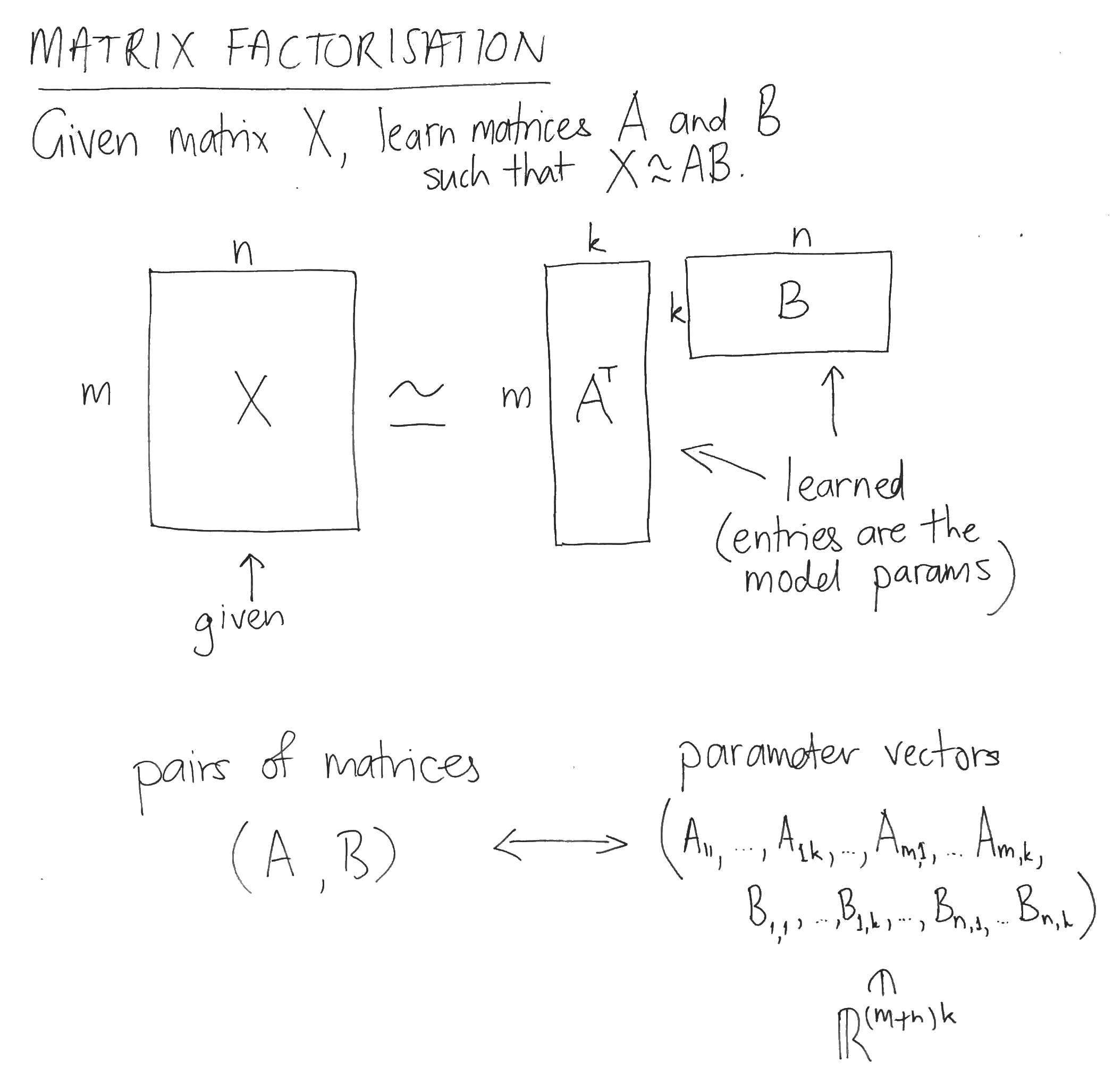The parameter space consists of the entries of the matrices $A$ and $B$. The hidden feature space, on the other hand, is the k-dimensional space containing the columns of $A$ and $B$.

### Error function

To train a matrix factorisation model using gradient descent, the model parameters are repeatedly updated using the gradient vector of the error function. An example error function $E$ could be

$E_X (A, B) = \sum_{i, j} {(X_{i, j} – (AB)_{i,j})^2}.$

Notice that this choice of error function doesn’t depend directly on the pair of matrices $(A, B)$, but rather only on their product $AB$, i.e. only on the approximation $AB$ of $X$. This is true of any error function $E_X$, because error functions depend only on inputs and outputs.

### Orthogonal transformations of the hidden feature space

Recall that orthogonal transformations of a space are just compositions of rotations and reflections about hyperplanes passing through the origin. Considered as matrices, orthogonal transformations are defined by the property that their product with their transpose gives the identity matrix. Using this property, it can be seen that an orthogonal transformation of the hidden feature space defines an orthogonal transformation of the parameter space by acting simultaneously on the column vectors of the matrices. If $O_k$ and $O_{(m+n)k}$ denote the groups of orthogonal transformations on the hidden feature space and the parameter space, respectively, then: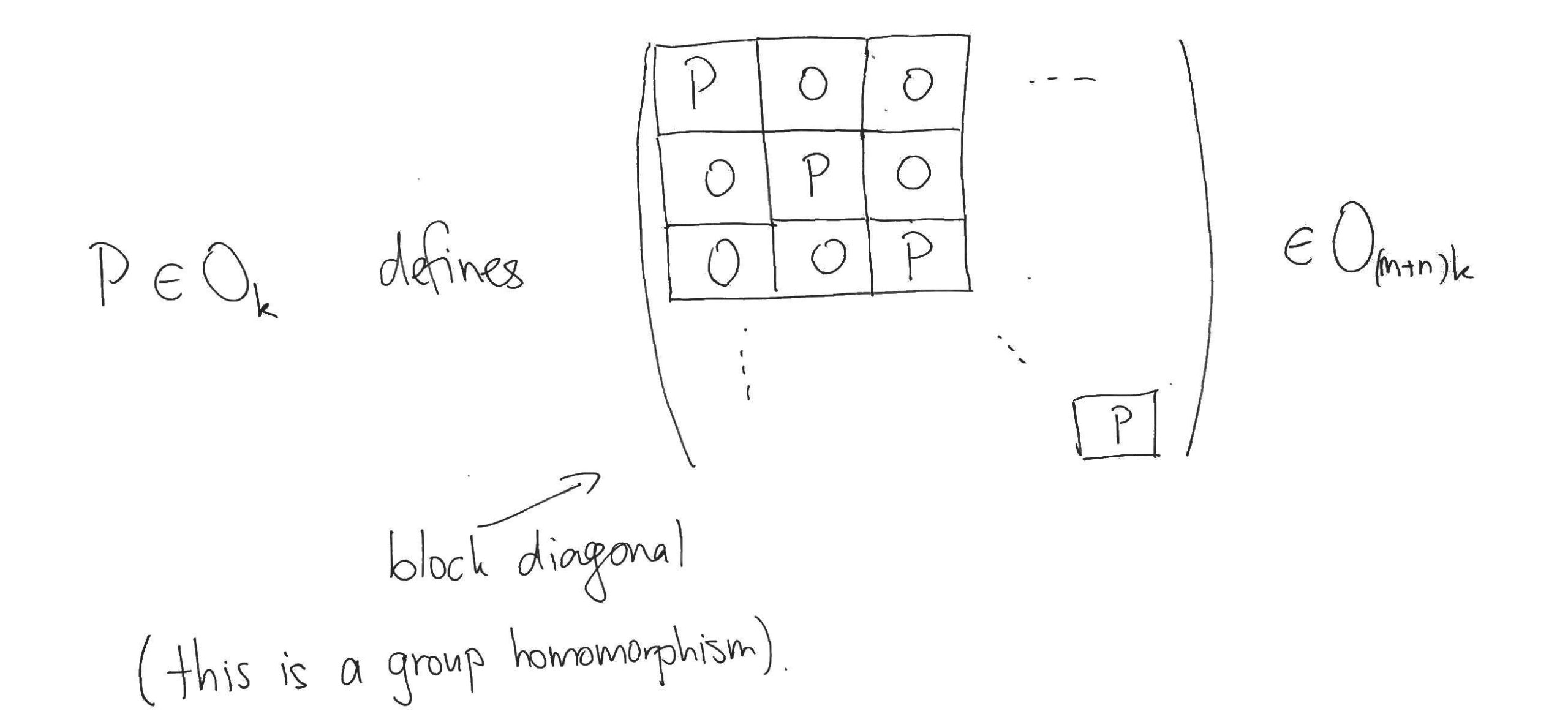### Contour lines of the gradient

The effect of this block-diagonal orthogonal transformation on the parameter space corresponds to multiplying the matrices $A$ and $B$ on the left by the orthogonal transformation $P$ of the feature space, i.e. it effects $(A, B) \mapsto (PA, PB)$. Notice that $(A, B)$ and $(PA, PB)$ yield the same approximation to the original matrix $X$, since:

$(PA)^T (PB) = (A^T P^T) (PB) = A^T (P^T P) B = AB.$

Thus $E_X (A, B) = E_X (PA, PB)$, so the orthogonal transformations $P$ of the hidden feature space trace out contour lines of $E_X$ in the parameter space. Now the gradient vector is always perpendicular to the contour line, so the sequence of points in the parameter space visited during gradient descent preserve the orientation of the hidden feature space set at initialisation (see here, for example). So if gradient descent of $E_X$ starting at the initial parameters $(A^{(0)}, B^{(0)})$ converges to the parameters $(A, B)$, and you’d prefer that it instead converged to $(PA, PB)$, then all you need to do is start the gradient descent over again, but this time with the initial parameters $(PA^{(0)}, PB^{(0)})$. We thus see that the matrices $(A, B)$ that our matrix factorisation model has learned are only determined up to an orthogonal transformation of the hidden feature space, i.e. up to a simultaneous transformation of their columns.

The above statements continue to hold in the case of stochastic gradient descent, where the error function $E_X$ is not fixed but rather defined by varying mini-tasks (an instance being e.g. word2vec). Such error functions still don’t depend upon hidden layer values, so as above their gradient vectors are perpendicular to the contour lines traced out by the orthogonal transformations of the hidden layer. Thus the updates performed in stochastic gradient descent also preserve the original orientation of the feature space.

### Initialisation

How likely is it that initial parameters, transformed via an orthogonal transformation as above, ever occur themselves as initial parameters? In order to conclude that the orientation of the co-ordinate system on the hidden layer is completely arbitrary, we need it to be precisely as likely. Thus if $\pi$ denotes the probability distribution on the parameter space from which the initial parameters are drawn, we require

$\pi ( (P A^{(0)}, P B^{(0)}) ) = \pi ( (A^{(0)}, B^{(0)}) ),$

for any initial parameters $( A^{(0)}, B^{(0)} )$ and any orthogonal transformation $P$ of the hidden feature space.

This is not the case with word2vec, where each parameter is drawn independently from a uniform distribution. However, it remains true that for any choice of initial parameters, there will still be any number of possible orientations of the co-ordinate system, but for some choices of initial parameters there is less freedom than for others.

GloVe performs weighted matrix factorisation with bias terms, so the above should apply. The weighting is just a modified error function, and the bias terms are not hidden features and so are left unmodified by its orthogonal transformations. Like word2vec, GloVe initialises each parameter with independent samples from uniform distribution, so there are no new problems there. The real problem with applying the above analysis to GloVe is that the implementation of Adagrad used makes the learning regime dependent on the choice of basis of the hidden feature space (see e.g. here). This doesn’t mean that the hidden unit activations of GloVe make sense, it just means that GloVe is less amenable to theoretical arguments like those above and needs to be considered empirically e.g. in the manner of Szegedy et al.

### Descartes’ headache: rotation of space is transpose to rotation of co-ordinate system

[I often speak of such-and-such depending upon the choice of co-ordinate system, and proceed to show this by rotating the space. The following explains why this is equivalent (via the transpose)].

You awaken in a two-dimensional landscape. In front of you are co-ordinate axes, fixed to the ground on a pivot. The landscape is featureless except for a tree and a well. You get bored, and fall asleep again. When you awaken, you notice that the relative arrangement of the co-ordinate axes to the tree and the well is different. What happened?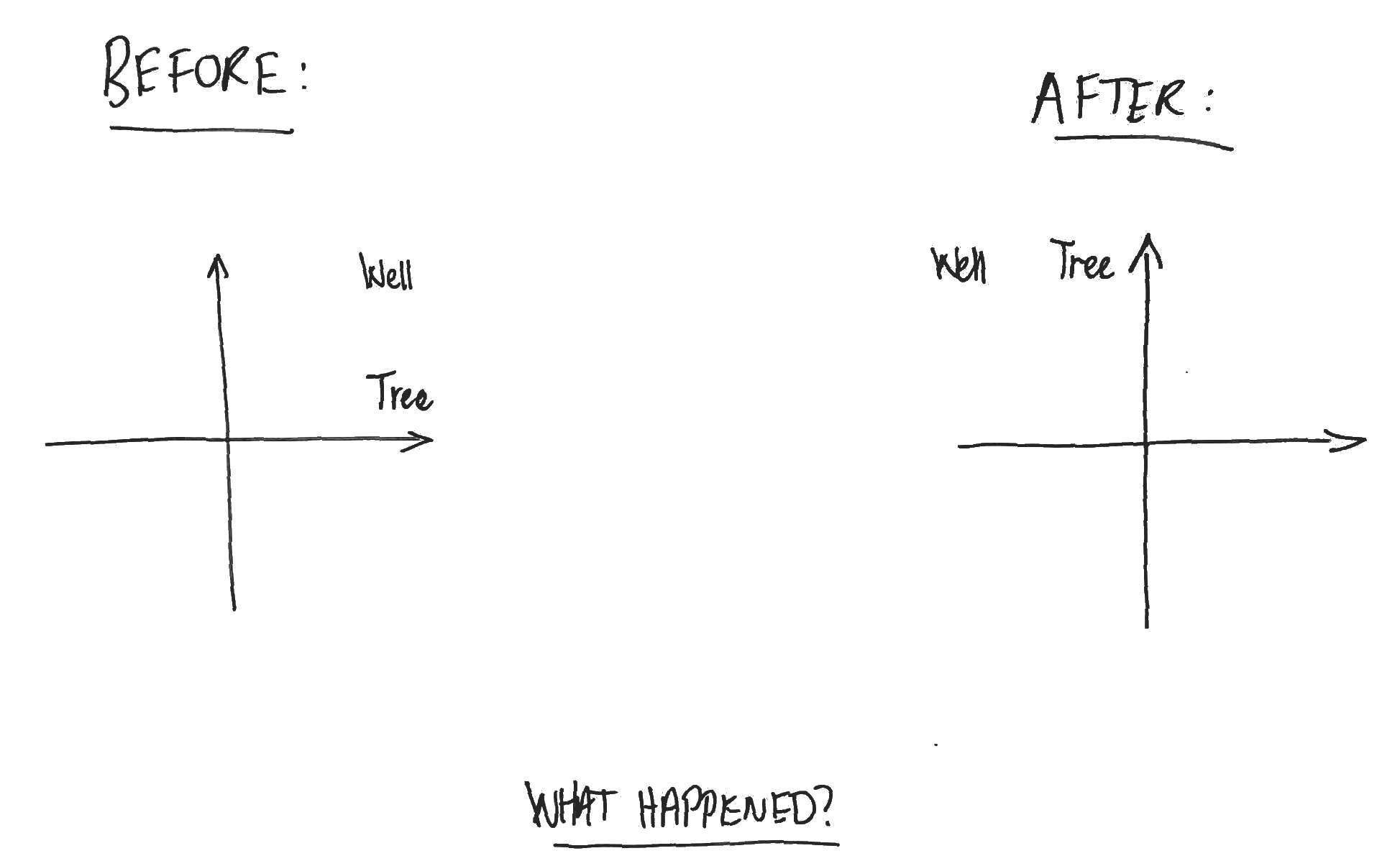You realise that you’ll never truly know what happened while you were asleep. Either (a) someone rotated the co-ordinate axes 90 degrees clockwise, or (b) someone rotated the world 90 degrees anti-clockwise about the pivot point of the axes.

Rotation of the space is equivalent (via the transpose) to rotation of the co-ordinate system. In fact, this is true more generally for orthogonal transformations and has a familiar mathematical expression. Let $V$ be an n-dimensional vector space with inner product $\langle \cdot , \cdot \rangle$ and let $e_i, i=1, .., n$ be an orthonormal basis. If $X$ is an orthogonal transformation of $V$, then for any point $v \in V$, we have
$$\displaystyle \langle Xv, e_i \rangle = \langle v, X^T e_i \rangle$$

for all $i=1, .. , n$. That is, the co-ordinates of the transformed point with respect to the original basis are the co-ordinates of the original point with respect to the transpose-transformed basis.

Adagrad is a learning regime that maintains separate learning rates for each individual model parameter. It is used, for instance, in GloVe (perhaps incorrectly), in LightFM, and in many other places besides. Below is an example showing that Adagrad models evolve in a manner that depends upon the choice of co-ordinate system (i.e. orthonormal basis) for the parameter space. This dependency is no problem when the parameter space consists of many one-dimensional, conceptually unrelated features lined up beside one another, because such parameter spaces have only one natural orientation of the co-ordinate axes. It is a problem, however, for the use of Adagrad in models like GloVe and LightFM. In these cases the parameter space consists of many feature vectors (of dimension, say, 100) concatenated together. A learning regime should not depend upon the arbitrary choice of orthonormal basis in this 100-dimensional feature space.

For feature spaces like these, I would propose instead maintaining a separate learning rate for each feature vector (for example, in the case of GloVe, there would be one learning rate per word vector per layer). The learning rate of a feature vector would dampen the initial learning rate by the accumulation of the squared norms of the previous gradient updates of the feature vector. The evolution of Adagrad would then be independent of the choice of basis in the feature space (as distinct from the entire parameter space). In the case of GloVe this means that a simultaneous rotation of all the word vectors in both layers during training does not alter the resulting model. This proposal would have the further advantage of greatly reducing the number of learning rates that have to be stored in memory. I don’t know if this proposal would have regret minimisation properties analogous to Adagrad. I haven’t read the original paper of Duchi et al. (2011), and what I am proposing might be subsumed there by the full-rank case (thanks to Alexey Rodriguez for pointing this out). Perhaps a block diagonal matrix could be used instead of a diagonal one.

Update: Minh + Kavukcuoglu seem to have adopted the same point of view in Learning word embeddings efficiently with noise-contrastive estimation (2013). Thanks to Matthias Leimeister for this.

We show that if the contour lines of a function are symmetric with respect to some rotation or reflection, then so is the evolution of gradient descent when minimising that function. Rotation of the space on which the function is evaluated effects a corresponding rotation of each of the points visited under gradient descent (similarly, for reflections).

This ultimately comes down to showing the following: if $f: \mathbb{R}^N \to \mathbb{R}$ is the differentiable function being minimised and $g$ is a rotation or reflection that preserves the contours of $f$, then

\begin{equation}\label{prop} \nabla |_{g(u)} f = g ( \nabla |_u f) \end{equation}

for all points $u \in \mathbb{R}^N$.

### Examples

We consider below three one-dimensional examples that demonstrate that, even if the function $f$ is symmetric with respect to all orthogonal transformations, it is necessary that the transformation $g$ be orthogonal in order for the property (\ref{prop}) above to hold.

### Short-time Fourier transform cheatsheet

I prepared the following one-page overview of the short-time Fourier transform for a recent talk, perhaps it’ll be useful to others. For justification of e.g. the conjugate symmetry of the Fourier coefficients or a discussion of aliasing, see here.

### The mathematics of the discrete Fourier transform

We aim to identify the assumptions that are implicit in the sampling of a continuous-time signal and in the subsequent application of the discrete Fourier transform (DFT). In particular, we consider the following questions:

• When does the sampling of periodic continuous-time signal result in a periodic discrete-time signal?
• When the resulting discrete-time signal is periodic, what is its frequency in samples/second?
• Which continuous-time frequencies coincide in discrete time, and what does the “frequency spectrum” in discrete-time look like?
• To which periodic discrete-time signals can the discrete Fourier transform be applied to without losing information?

We furthermore show that the DFT interchanges point-wise and convolution products in the time- and frequency- domains, and thereby express the DFT to Pontryagin duality for finite cycle groups.

I talked about the above (skipping many details!) at a recent talk.

### Feature scaling and non-negative matrix factorisation

Non-negative matrix factorisation (NMF) is a dimension reduction technique that is commonly applied in a number of different fields, for example:

• in topic modelling, applied to the document x word matrix;
• in speech processing, applied to the matrix of magnitude spectrograms of framed audio;
• in recommendation systems, applied to the user x item interaction matrix.

Due to its non-negativity constraint, it has the wonderful property of decomposing a objects as an additive combination of (often very meaningful) parts. However, as with all unsupervised learning tasks, it is sensitive to the relative scale of different features.

The fundamental problem is that the informativeness of a feature need not be related to its scale. For example, when processing speech, the highest-energy components of a magnitude spectrogram are those of the least perceptual importance! So when NMF decides which information to discard into order to achieve a low-rank factorisation that minimises the error function, it can be the signal, not the noise, that is sacrificed. This problem is not unique to NMF, of course: PCA retains those dimensions of the sample cloud that have the greatest variance.

It is in general better to learn a feature representation jointly with the downstream task, so that the model learns to scale features according to their informativeness for the task. If NMF is for some reason still desirable, however, it is possible to better control the information loss by choosing an appropriate measure of the matrix factorisation error.

There are three common error functions used in NMF (all of which Bregman divergences): squared Euclidean, Kullback-Leibler (KL) and Itakura-Saito (IS). These are respectively quadratic, linear and invariant with respect to the feature scale. Thus, for example, NMF with the Euclidean error function gives strong preference to high-energy features, while NMF with the IS error function is agnostic to feature scale.

### Convergence rate of gradient descent

These are notes from a talk I presented at the seminar on June 22nd. All this material is drawn from Chapter 7 of Bishop’s Neural Networks for Pattern Recognition, 1995.

In these notes we study the rate of convergence of gradient descent in the neighbourhood of a local minimum. The eigenvalues of the Hessian at the local minimum determine the maximum learning rate and the rate of convergence along the axes corresponding to the orthonormal eigenvectors.

See the eigendecomposition of real, symmetric matrices for the linear algebra preliminaries.

### Skipgram isn't Matrix Factorisation

The paper Neural Word Embeddings as Implicit Matrix Factorization of Levy and Goldberg was published in the proceedings of NIPS 2014 (pdf).  It claims to demonstrate that Mikolov’s Skipgram model with negative sampling is implicitly factorising the matrix of pointwise mutual information (PMI) of the word/context pairs, shifted by a global constant.  Although the paper is interesting and worth reading, it greatly overstates what is actually established, which can be summarised as follows:

Suppose that the dimension of the Skipgram word embedding is at least as large as the vocabulary.  Then if the matrices of parameters $(W, C)$ minimise the Skipgram objective, and the rows of $W$ or the columns of $C$ are linearly independent, then the matrix product $WC$ is the PMI matrix shifted by a global constant.

This is a really nice result, but it certainly doesn’t show that Skipgram is performing (even implicitly) matrix factorisation.  Rather it shows that the two learning tasks have the same global optimum  – and even this is only shown when the dimension is larger than the vocabulary, which is precisely the case where Skipgram is uninteresting.

### The linear independence assumption

The authors (perhaps unknowingly) implicitly assume that the word vectors on one of the two layers of the Skipgram model are linearly independent.  This is a stronger assumption than what the authors explicitly assume, which is that the dimension of the hidden layer is at least as large as the vocabulary.  It is also not a very natural assumption, since Skipgram is interesting to us precisely because it captures word analogies in word vector arithmetic, which are linear dependencies between the word vectors!  This is not a deal breaker, however, since these linear dependencies are only ever approximate.

In order to see where the assumption arises, first recall some notation of the paper:

The authors consider the case where the negative samples for Skipgram are drawn from the uniform distribution $P_D$ over the contexts $V_C$, and write

for the log likelihood.  The log likelihood is then rewritten as another double summation, in which each summand (as a function of the model parameters) depends only upon the dot product of one word vector with one context vector:

The authors then suppose that the values of the parameters $W, C$ are such that Skipgram is at equilibrium, i.e. that the partial derivatives of $l$ with respect to each word- and content-vector component vanish.  They then assume that this implies that the partial derivatives of $l$ with respect to the dot products vanish also.  To see that this doesn’t necessarily follow, apply the chain rule to the partial derivatives:

This yields systems of linear equations relating the partial derivatives with respect to the word- and content- vector components (which are zero by supposition) to the partial derivatives with respect to the dot products, which we want to show are zero.  But this only follows if one of the two systems of linear equations has a unique solution, which is precisely when its matrix of coefficients (which are just word- or context- vector components) has linearly independent rows or columns.  So either the family of word vectors or the family of context vectors must be linearly independent in order for the authors to proceed to their conclusion.

Word vectors that are of dimension the size of the vocabulary and linearly independent sound to me more akin to a one-hot or bag of words representations than to Skipgram word vectors.

### Skipgram isn’t Matrix Factorisation (yet)

If Skipgram is matrix factorisation, then it isn’t shown in this paper.  What has been shown is that the optima of the two methods coincide when the dimension is larger that the size of the vocabulary. Unfortunately, this tells us nothing about the lower dimensional case where Skipgram is actually interesting.  In the lower dimensional case, the argument of the authors can’t be applied, since it is then impossible for the word- or context- vectors to be linearly independent.  It is only in the lower dimensional case that the Skipgram and Matrix Factorisation are forced to compress the word co-occurrence information and thereby learn anything at all.  This compression is necessarily lossy (since there are insufficient parameters) and there is nothing in the paper to suggest that the two methods will retain the same information (which is what it means to say that the two methods are the same).

### Appendix: Comparing the objectives

To compare Skipgram with negative sampling to MF, we might compare the two objective functions.  Skipgram maximises the log likelihood $l$ (above). MF, on the other hand, typically minimises the squared error between the matrix and its reconstruction:

The partial derivatives of $E$, needed for a gradient update, are easy to compute:

Compare these with the partial derivatives of the Skipgram log-likehood $l$, which can be computed as follows:

### Softmax parameterisation and optimisation

The softmax function provides a convenient parameterisation of the probability distributions over a fixed number of outcomes. Using the softmax, such probability distributions can be learned parametrically using gradient methods to minimise the cross-entropy (or equivalently, the Kullback-Leibler divergence) to observed distributions.  This is equivalent to maximum likelihood learning when the distributions to be learned are one-hot (i.e. we are learning for a classification task). In the notes below, the softmax parameterisation and the gradient updates with respect to the cross entropy are derived explicitly.

This material spells out section 4 of the paper of Bridle referenced below, where the softmax was first proposed as an activation function for a neural network. It was in this paper that softmax was named, moreover. The name contrasts the outputs of the function with those of the “winner-takes-all” function, whose outputs are one-hot distributions.

### References

Bridle, J.S. (1990a). Probabilistic Interpretation of Feedforward Classification Network Outputs, with Relationships to Statistical Pattern Recognition. In: F.Fogleman Soulie and J.Herault (eds.), Neurocomputing: Algorithms, Architectures and Applications, Berlin: Springer-Verlag, pp. 227-236.

### Improving Pairwise Learning for Item Recommendation from Implicit Feedback 2014

Steffen Rendle and Christoph Freudenthaler (University of Konstanz), WSDM 2014.
The authors present a modification of the Bayesian Pairwise Ranking (BPR) for implicit feedback (i.e. one class) recommendation datasets in which the negative samples are drawn according both to the models current belief and the user/context in question (“adaptive oversampling“). They show that the prediction accuracy of BPR models trained with adaptive oversampling matches that of BPR models trained with uniform sampling but that convergence is 10x-20x faster.
Consider the problem of recommending items to users (or more generally: contexts, e.g. user on a particular page).  The observed data consists then of context-item pairs $(c, i)$, where item $i$ was the choice made in context $c$.  The authors work in the context of pairwise learning, which amounts to a binary classification task where context-item-item triples $(c, i, j)$ are labelled as true i.f.f. item $i$ was chosen in context $c$ but item $j$ is not: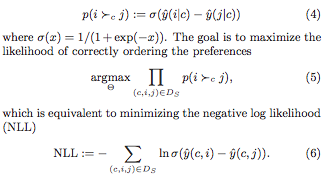where $\hat{y}$ is a scoring function (e.g. the dot product of the context- and the item- latent vectors, for matrix factorisation).  It is infeasible to consider all the negative examples $j$, so how should we choose which to consider?

### Negative sampling from static distributions

We could draw negative examples from the uniform distribution over all items, or instead from the observed distribution over all items (i.e. by popularity).  Both are inexpensive to perform and easy to implement.  However:
• Uniform sampling tends to yield uninformative samples, i.e. those for which the probability of being incorrectly labelled is very likely already low: popular items are precisely those that appear often as positive examples (and hence tend to be highly ranked by the model), while a uniformly-drawn item is likely to be from the tail of the popularity distribution (so likely lowly ranked by the model).
• Sampling according to popularity is demonstrated by the authors to converge to inferior solutions.
The authors point out that these sampling schemes depend neither upon the current context (user) nor the current belief of the model.  This contrasts with their own method, adaptive over-sampling.

The authors propose a scheme in which the negative samples chosen are those that the model would be likely to recommend to the user in question, according to its current state.  In this sense it is reminiscent of the Gibbs sampling used by restricted Boltzmann machines.
Choosing negative samples dependent on the current model and user is computationally expensive if performed in the naive manner. The authors speed this up by working with the latent factors individually, and only periodically re-computing the ranking of the items according to each latent factor.  Specifically, in the case of matrix factorisation, when looking for negative samples for a context $c$, a negative sample is chosen by:
1. sampling a latent factor $l$ according to the absolute values of the latent vector associated to $c$ (normalised, so it looks like a probability distribution);
2. sampling an item $j$ that ranks highly for $l$.  More precisely, sample a rank $r$ from a geometric distribution over possible ranks, then find the item that has rank $r$ when the $l$th coordinates of the item latent vectors are compared.

(We have ignored the sign of the latent factor.  If the sign is negative, one choses the rth-to-last ranked item).  The ranking of the items according to each latent factor is precomputed periodically with a period such that the extra overhead is independent of the number of items (i.e. is a constant).

### Problems with the approach

The samples yielded by the adaptive oversampling approach depend heavily upon the choice of basis for the latent space.  It is easy to construct examples of where things go wrong:

Non-negativity constraints would solve this.  Regularisation would also deal with this particular example – however regularisation would complicate the expression of the scoring function $\hat y$ as a mixture (since you need to divide though by $Z_c$.

Despite these problems, the authors demonstrate impressive performance, as we’ll see below.

### Performance

The authors demonstrate that their method does converge to solutions slightly better than those given by uniform sampling, but twenty times faster.  It is also interesting to note that uniform sampling is vastly superior to popularity based sampling, as shown in the diagrams below.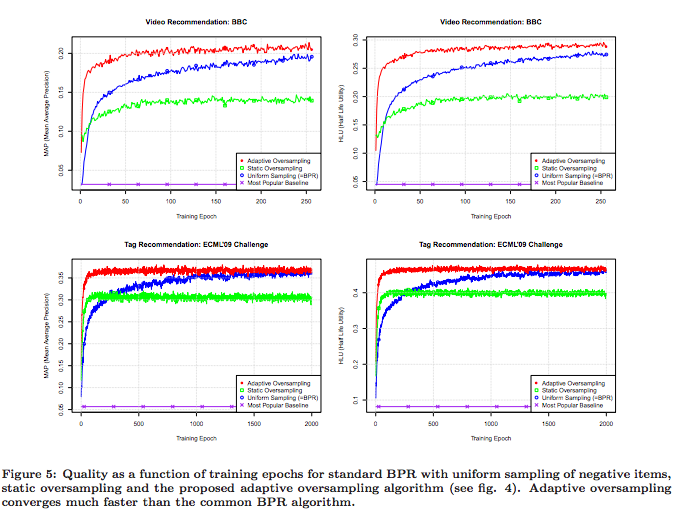Note that a single epoch of the adaptive oversampling takes approximately 33% longer than a single epoch of uniform sampling BPR.

### Implementation

According to the paper, the method is implemented in libFM, a C++ software package that Rendle has published.  However, while I haven’t looked exhaustively, I can’t see anything in that package about the adaptive oversampling (nor in Rendle’s other package, MyMediaLite).

Word2vec with negative sampling learns a word embedding via binary classification task, specifically, “does the word appear in the same window as the context, or not?”.  As in the case of implicit feedback recommendation, the observed data for word2vec is one-class (just a sequence of words).  Interestingly, word2vec samples from a modification of the observed word frequency distribution (i.e. of the “distribution according to popularity”) in which the frequencies are raised to the $0.75$th power and renormalised.  The exponent was chosen empirically.  This raises two questions:

1. Would word2vec perform better with adaptive oversampling?
2. How does BPR perform when sampling from a similarly-modified item popularity distribution (i.e. raising to some exponent)?

### Corrections

Corrections and comments are most welcome. Please get in touch either via the comments below or via email.

### WARP loss for implicit-feedback recommendation

We consider the “Weighted Approximate-Rank Pairwise-” (WARP-) loss, as introduced in the WSABIE paper of Weston et. al (2011, see references), in the context of making recommendations using implicit feedback data, where it has been shown several times to perform excellently.  For the sake of discussion, consider the problem of recommending items $i$ to users $u$, where a scoring function $f_u(i)$ gives the score of item $i$ for user $u$, and the item with the highest score is recommended.

WARP considers each observed user-item interaction $(u, i)$ in turn, choses another “negative” item $i’$ that the model believed was more appropriate to the user, and performs gradient updates to the model parameters associated to $u$, $i$ and $i’$ such that the models beliefs are corrected.  WARP weights the gradient updates using (a function of) the estimated rank of item $i$ for user $u$.  Thus the updates are amplified if the model did not believe that the interaction $(u, i)$ could ever occur, and are dampened if, on the other hand, if the interaction is not surprising to the model. Conveniently, the rank of $i$ for $u$ can be estimated by counting the number of sample items $i’$ that had to be considered before one was found that the model (erroneously) believed more appropriate for user $u$.

### Minimising the rank?

Ideally we would like to make updates to the model parameters that minimised the rank of item $i$ for user $u$.  Previous work of Usunier (one of the authors) showed that the precision at k was best optimised when the logarithm of the rank was minimised.  (to read!)

The problem with the rank is that, while it does depend on the model parameters, this dependence is not continuous (the rank being integer valued!).  So it is not possible to speak of gradients.  So what is to be done instead?  The approach of the authors is to derive a differentiable approximation to the logarithm of the rank, and to minimise this instead.

### Derivation: approximating the (log of the) rank

WARP has been shown several times to perform very well for implicit feedback recommendation.  However, the derivation of the approximation of the log of the rank used in WARP, as outlined in the WSABIE paper, is nonsense.  I can only think that the authors arrived at WARP in another way.  Let’s look at it more closely.  In the following:

• $f_u (i)$ is the score assigned by the model to item $i$ for user $u$.
• $L$ is some function that defines the error as a function of the rank.  In the WSABIE paper, $L(k) = \sum_{j=1}^k \frac{1}{j}$ is approximately the natural logarithm (for the derivation below, however, it doesn’t matter what $L$ is)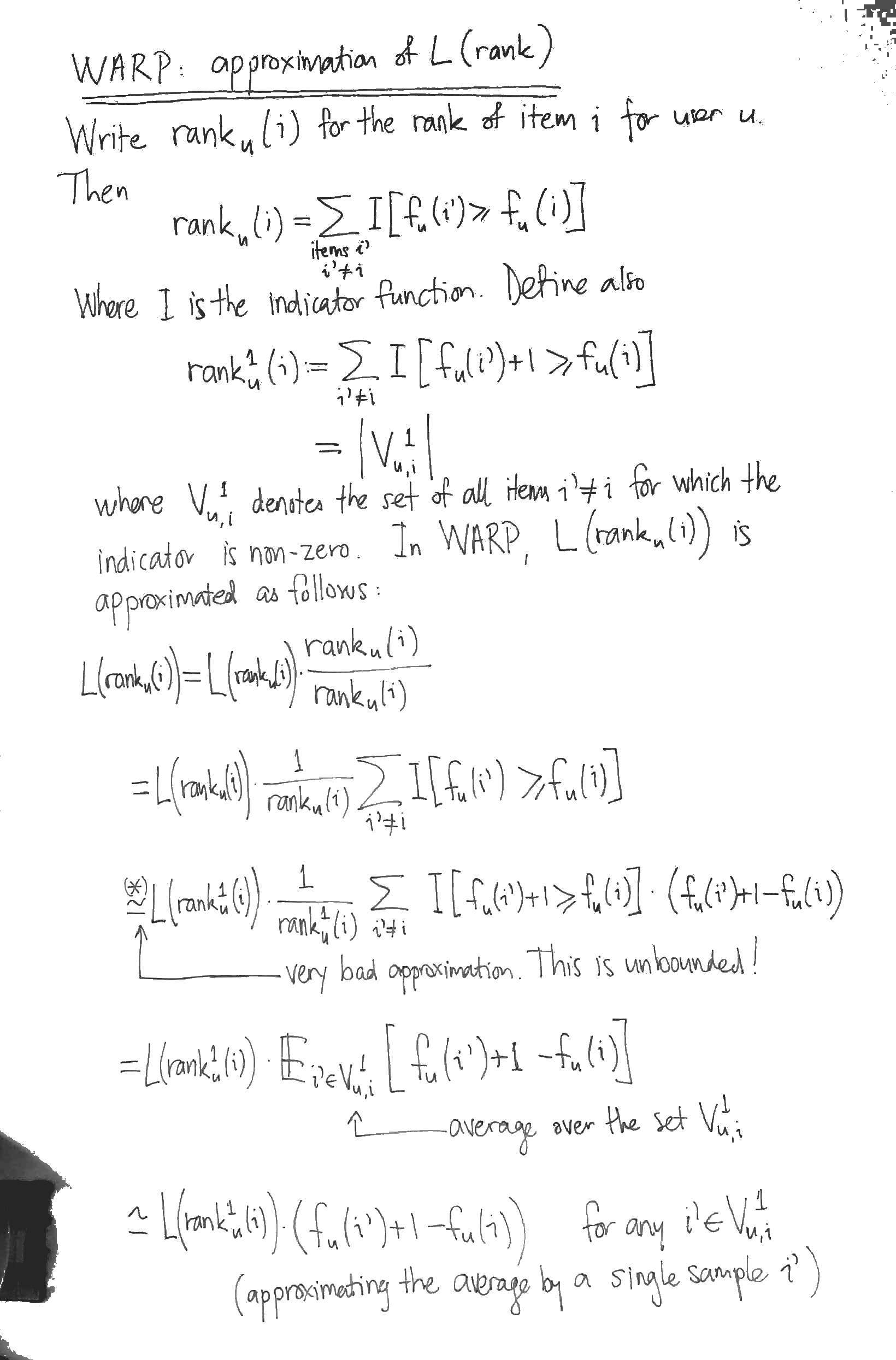The most obvious problem with the derivation is the approximation marked with an asterix (*).  At this step, the authors approximate the indication function $I[x > y]$ by $I[x > y] \cdot (x – y + 1)$.  While the latter is familiar as the hinge loss from SVMs, it is (begin unbounded!) a dreadful approximation for the indicator $I[x > y]$.  It seems to me that the sigmoid of the difference of the scores would be a much better differentiable approximation to the indicator function.

To appreciate why the derivation is nonsense, however, you have to notice that the it has nothing to do with $L$.  That is, the derivation above would yield an approximation for $L$, whatever $L$ happened to be, even a constant function.

### Optimisation

WARP considers each observed interaction $(u, i)$ in turn, repeatedly sampling items $i’$ from the uniform distribution over all items until it finds one in $V_{u, i}^1$, i.e. until it finds an item $i’$ whose score for the user $u$ is at worst 1 less than the score of the observed interaction.  For this triple $(u, i, i’)$, it performs gradient updates to minimise:

$\displaystyle L( \text{rank}_u^1 (i) ) \cdot (f_u (i’) + 1 – f_u (i))$

The naive approach to computing $\text{rank}_u^1 (i)$ is to calculate all the scores for the given user in order to determine the rank of the item $i$.  WARP performs a nice trick to do much better: it estimates $\text{rank}_u^1 (i)$ by counting how many candidate negative items $i’$ it had to consider before finding one in $V_{u, i}^1$.  This yields

$\displaystyle \text{rank}_u^1 (i) \approx \frac{\text{total number of items} – 1}{\text{number of i’ we had to draw}}$

However it is still the case that $L(\text{rank}_u^1 (i))$ is not differentiable.  So when we compute the gradients, this quantity has to be treated as a constant. Thus it simply becomes a weighting applied to the gradient of the difference of the scores (hence the name WARP, I guess).

### WARP optimises for item to user recommendations

With its negative sampling technique, WARP optimises for recommending items to a user.  For instance, the problem of recommending users to items (so, transposing the interaction matrix) is not trained for.  I wonder if some extra uplift could be obtained by training for both problems simultaneously.

### Normalising for the total number of items

With the optimisation stated as above, the learning rate will need to be re-tuned for datasets that have different numbers of items, since the gradient weighting $L( \text{rank}_u^1 (i) )$ is ranges from $L(0)$ to $L(\text{total number items})$.  It would make more sense to weigh the gradient updates by:

$\displaystyle \frac{L( \text{rank}_u^1 (i) )}{L(\text{total number items})}$

which ranges between 0 and 1.

### Implementations

There are two implementations of WARP for recommendation that I know of, both in Python:

• LightFM, written by Maciej Kula.  Works well.  Also implements BPR with uniform sampling and WARP k-OS (which I’ve not investigated yet).
• MREC, written by Levy and Jack at Mendeley, has a matrix factorisation recommender trained using WARP.  I’ve not tried this one out yet.

### References

Jason Weston, Samy Bengio and Nicolas Usunier, Wsabie: Scaling Up To Large Vocabulary Image Annotation, 2011, (PDF).

### Metadata Embeddings for User and Item Cold-start Recommendations

Maciej Kula (Lyst)
CBRecSys 2015 (arxiv)

Kula presents a model for cold start recommendation, which he calls “LightFM”.  Users and items are considered as sets of binary features. For example:

$\text{alice} = \{ \text{domain}:\text{gmail} \}$

$\text{itemXYZ} = \{\text{description}:\text{pleated}, \text{description}:\text{skirt}, \text{tag}:\text{chanel} \}.$

Each of these features (e.g. each tag, each word and each email domain) corresponds to a parameter vector and a bias term.  A user vector (or item vector) is then the sum of the vectors associated to its constituent features.  Similarly, a user (item) bias term is just the sum of the bias terms associated to its features.

The probability $\hat{r}(u, i)$ of an interaction between a user $u$ and an item $i$ is modelled as the sigmoid of the dot product of the user vector and the item vector, along with the bias terms associated with the user and the item:

$\hat{r}(u, i) := \sigma (vec(u) \cdot vec(i) + bias(u) + bias(i))$

The model is trained on a set $S_{+}$ of user-item pairs observed as having interacted, and on a set $S_{-}$ of user-item pairs that were not observed to have interacted (in the case of implicit feedback recommendation) or to have interacted negatively (in the case of explicit feedback recommendation).  Specifically, these interactions and non-interactions are assumed independent and the likelihood

$\displaystyle L = \prod_{(u, i) \in S_{+}} \hat{r}(u,i) \cdot \prod_{(u, i) \in S_{-}} (1 – \hat{r}(u,i))$

### Trivial featurisation gives matrix factorisation

Note that users (or items) can be featurised trivially using their ids.   We create one user feature for each user id, so that the user-feature matrix is the identity matrix.  In this case, we have a separate parameter vector for each user.  If we do this for both users and items, then the model is just a (sigmoid-) factorisation of the user-item interaction matrix. This is then the case of Johnson’s logistic matrix factorization.

### Evaluation

Performance is evaluated on MovieLens for explicit feedback recommendation and on CrossValidated (one of the StackExchange websites) for implicit feedback recommendation.  In both cases, warm- and cold-start scenarios are tested.  Warm start is tested by holding out interactions in such a way that every item and every user is still represented in the training interaction data.  Cold start is tested by holding out all interactions for some items.  Model accuracy is measured by considering each user in the set of test interactions, considering the binary classification task of labelling each item as having been interacted with or not and then measuring the area under the curve of the associated ROC curve.  The mean is that taken over all users in the test set.

LightFM seems to perform well in both cold and warm start scenarios.

### Engineering Notes

Kula included some interesting notes on the production use of LightFM at Lyst.  Training is incremental with model state stored in the database.

### Implementation and Examples

Available on GitHub and extensively documented.  Written in Cython.  In addition to the logistic loss used above, Bayesian Personalised Ranking and WARP are supported.

### Parameterising the Mahalanobis distances for metric learning

Below are the notes I made to prepare for a short talk given at our seminar on learning distance metrics, and the Mahalanobis distances in particular. We show that the Mahalanobis distances can be parameterised by the positive semidefinite (PSD) matrices or alternatively (in a highly redundant way) by all matrices. The set of PSD matrices is convex, but in order to perform gradient descent to optimise the objective function, we need to perform a costly projection after each update involving the singular value decomposition.

We note along the way that a Mahalanobis distance is nothing more than the Euclidean distance after applying a linear transform to the data.

The example of the 2×2 PSD matrices is worked out in detail here.

What’s here documents my first steps. What I really discovered is that metric learning is a research domain in its own right, and that a great deal of work has been done. There is an excellent survey by Bellet et al. (2013) that covers everything I have said in the first two of its sixty pages.

### Visualising the set of 2×2 positive semidefinite matrices

Recall that a symmetric matrix $M \in \mathbb{R}^{n \times n}$ is called positive semidefinite (“PSD”) if, for any $x \in \mathbb{R}^n$, we have $x^{T} M x \geqslant 0$. Positive semidefinite matrices occur, for instance, in the study of bilinear forms and as the Gram (or covariance) matrices in probability theory. In the case where $n = 2$, the space of symmetric matrices is 3-dimensional, and we can actually draw the subset of all positive semidefinite matrices – it looks like the bow of a ship.

It is clear that in the case illustrated below, the PSD matrices form a convex subset. It is easy to show this in general, by observing that the set of all PSD matrices is closed under addition and multiplication by non-negative scalars. The convexity of this set is crucial for the fitting of Mahalanobis distances in metric learning, which is how I got interested PSD matrices in the first place.

### Does vector direction encode word frequency?

In a paper with Adriaan Schakel, we presented controlled experiments for word embeddings using pseudo-words. Performing these experiments in the case of word2vec CBOW showed that, in particular, the vector direction of any particular word changed only moderately when the frequency of the word was varied. Shortly before we released the paper, Schnabel et al presented an interesting paper at EMNLP, where (amongst other things), they showed that it was possible to distinguish rare from frequent words using logistic regression on the normalised word vectors, i.e. they showed that vector direction does approximately encode coarse (i.e. binary, rare vs. frequent) frequency information.  Here, I wanted to quickly report that the result of Schnabel et al. holds for the vectors obtained from our experiments, as they should. Below, I’ll walk through exactly what I checked.

I took the word vectors that we trained during our experiments. You can check our paper for a detailed account. In brief, we trained a word2vec CBOW model on popular Wikipedia pages with a hidden layer of size 100, negative sampling with 5 negative samples, a window size of 10, a minimum frequency of 128, and 10 passes through the corpus. Sub-sampling was not used so that the influence of word frequency could be more clearly discerned. There were 81k unigrams in the vocabulary. Then:

1. the word vectors were normalised so that their (Euclidean-) length was 1.
2. the frequency threshold of 5000 was chosen (somewhat arbitrarily) to define the boundary between rare and frequent words. This gave 8428 “frequent” words. A random sample of the same size of the remaining “rare” words was then chosen, so that the two classes, “rare” and “frequent”, were balanced. This yielded approximately 17k data points, where a data point is a normalised word vector labelled with either “frequent” (1) or “rare” (0).
3. the data points were split into training- and test- sets, with 70% of the data points in the training set.
4. a logistic regression model was fit on the training set. An intercept was fit, but this boosted the performance only slightly. No regularisation was used since the number of training examples wass high compared to the number of parameters.
5. The performance on the test set was assessed by calculating the ROC curve on the training and test sets and the accuracy on the test set.

Model performance
Consider the ROC curve below. We see from that fact that the test curve approximately tracks the training curve that the model generalises reasonably to unseen data. We see also from the closeness of the curves to the axes at the beginning and the end that the model is very accurate in detecting frequent words when it gives a high probability (bottom left of the curve) and at detecting infrequent words when it gives a low probability (top right).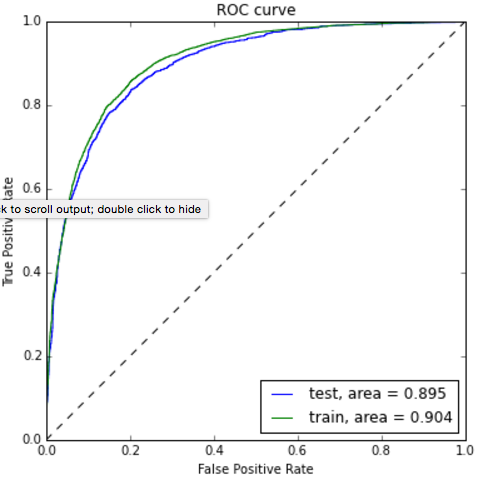The accuracy of the model on the test set was 82%, which agrees very nicely with what was reported in Schnabel et al., summarised in the following image: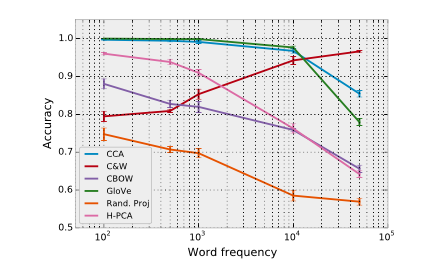The training corpus and parameters of Schnabel, though not reported in full detail (they had a lot of other things to report), seem similar. We know that their CBOW model was 50 dimensional, had a vocabulary of 103k words, and was trained on the 2008 Wikipedia.

### Limiting Distributions of Markov Chains

Below are the notes I prepared for a talk that I gave at our seminar on limiting distributions for (finite-state, time-homogeneous) Markov chains, drawing on PageRank as an example. We see in particular how the “random teleport” possibility in the PageRank random walk algorithm can be motivated by the theoretical guarantees that result from it: the transition matrix is both irreducible and aperiodic, and iterated application of the transmission matrix converges more rapidly to the unique stationary distribution.

Update: that the limit of the average time spent is the reciprocal of the expected return time can be proved using the renewal reward theorem (thank you Prof. Sigman!)

### A Bayesian Personalised Ranking Example: Factor Models for Recommending Given Names

Immanuel Bayer and Steffen Rendle

ECML PKDD Discovery Challenge 2013 (offline track).

This paper provides an interesting example of using factorisation machines for implicit feedback via the Bayesian personalised ranking (BPR) optimisation criterion.

The challenge was to recommend first names (e.g. to soon-to-be parents). Participants were provided with the browsing history of users on the name selection website Nameling. So the items to be recommended are names.

Users are considered together with their browsing history, consisting of the name they looked at last and the list of all the names they looked at up to the time $t$ in question. The combination of a user (in this complete sense) at time $t$ and a candidate name is vectorised as follows: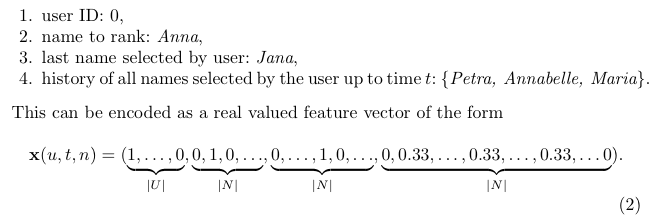The (order 2) factorisation machine assigns a score to the combination as follows:where $p$ is the rank of this vectorisation, $w_0 \in \mathbb{R}$, $w \in \mathbb{R}^p$ and $V \in \mathbb{R}^{p \times k}$ are model parameters to be learned. These parameters are learned via stochastic gradient ascent of the following pair-wise learning objective: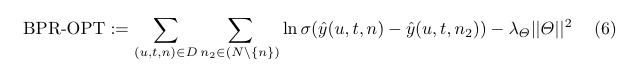where $D$ is the set of (user u, time t, name n) tuples where u has browsed n before time t, $N$ is the set of all possible names and $\sigma$ is the sigmoid function. Only a single name is chosen from $N \setminus \{ n \}$ for each update. These negative samples are drawn according to their estimated rank (this part is quite difficult to do efficiently).

In the above, we have purposefully omitted the “prefix smoothing” step, since we are mainly interested in the a simple factorisation machine example. The details are in the paper.

Remarks
This recommender did very well in the challenge. However, I don’t find the examples given in the paper very impressive (though I have not seen the examples given by others). My suspicion is that the FM approach is very strong, but that there is no good way to make first name recommendations using the provided dataset. A more effective dataset would be e.g. first name x product interaction on a large e-commerce site. This would do a much better job of capturing the social meaning of names, but could go out of date very quickly.

### Musings on "adjectives as matrices"

The advantage of considering (e.g.) adjectives as transformations rather than points in space is that these transformations can be applied in unseen combinations. This counters one of Chomsky’s objections to statistical modelling of language, that is, that language is effectively infinite, whereas language models are trained on only a finite amount of data (so are humans, but humans are supposed to be born with a universal grammar). The case, considered by Baroni et al., of adjective as linear transform has a couple of disadvantages, however. The first that there are a large number of parameters to be learnt for each adjective, the second being that it doesn’t capture the near commutativity of adjectives, i.e. in most cases adjectives can be applied to a noun in different orders without significantly changing the meaning.

I can think of several approaches for enforcing the commutativity of adjective matrices:

1. simply using diagonal matrices (this reduces to one of the approaches already considered), or
2. penalising the off-diagonal elements via regularisation, or
3. interleaving existing parameter updates with updates that penalise (co-occurring?) adjective matrices for not commuting with one another, e.g. using the gradient of the matrix commutator $AB – BA$

### (Linear) Maps of the Impossible: Capturing semantic anomalies in distributional space

Eva Maria Vecchi, Marco Baroni and Roberto Zamparelli.

Presented at the workshop “Distributional Semantics and Compositionality” (2011) PDF

The authors attempt to use distributional models to distinguish between acceptable and “semantically deviant” adjective-noun combinations (an example of this distinction is given by “blue rose” vs “residential steak”). They hypothesise in particular that the length of the vector representation of the adjective-noun combination is an indication of its acceptability. Their reasoning for this hypothesis assumes that directions and in particular axes are interpretable in distributional models (this does not apply in the case of word2vec, at least). They further hypothesise that the combination will be spatially isolated with respect to the cosine similarity.

The distributional representation is derived from a POS-tagged and lemmatised corpus by considering sentence-internal co-occurrence between the vocabulary as a whole and the 10k most frequent nouns, verbs and adjectives, transformed via the “local mutual information” measure and reduced to rank 300 using PCA.

Different methods of transforming the noun representation using the adjective to obtain the adjective-noun combination are studied and the results are evaluated against human judgements of semantic deviance.

### Collaborative Filtering for Implicit Feedback Datasets

Hu, Koren and Volinsky (AT&T, Yahoo!), 2008.

A well-written paper.

PDF

The authors give a good description of the distinctions between explicit and implicit feedback datasets, pointing out in particular that:

1. implicit feedback data is inherently noisy, since a user might decide that they do not like an item after viewing it — interaction does not necessarily indicate interest.
2. the numerical value in explicit feedback indicates preference whereas in the implicit case indicates confidence.

The authors describe their model as being based on SVD, but this is not accurate, since they weight squared difference summands in the cost function according to a confidence value (which is proportional to the number of interactions for that user-item pair).

The input matrix is the user-item matrix.

Optimisation is via alternating least squares.

Their evaluation metric is percentile rank based.

Their model, which we’ll call “weighted SVD” (they speak of “confidence intervals”) compares favourably with the baseline popularity method and also with an old-school item-based neighbourhood method, in terms of the expected percentile rank (Figure 1). Interestingly, the differences are less marked when the probability that a desired item is in the top (say) 1% is considered (Figure 2).

The unweighted SVD on the user-item matrix is shown to perform terribly, with a significant but insufficient improvement obtained with regularisation.

### Logistic Matrix Factorization for Implicit Feedback Data

Christopher Johnson, Spotify, 2014

PDF

A new matrix factorisation model for behavioural recommendation in the case of implicit feedback is presented.

User-item interactions are encoded in a non-negative interaction matrix. The question as to whether a user-item interaction occurred is then treated as a problem of binary classification. User-item pairs for which an interaction has occurred are regarded as positive outcomes with confidence in constant proportion to the value in the interaction matrix, while the absence of an interaction is regarded as a negative outcome. This binary classification is task is then leveraged to train user- and item-vectors. These vectors reside in dually paired spaces. The dot-product of the vectors, combined with user- and item- bias terms, is then fed into the sigmoid function. What we are really doing is looking for a low-rank approximation to a bilinear form via the sigmoid function.

Confidence values (proportional to the values in the interaction matrix) are used as powers of their corresponding factor in the maximum likelihood function. The constant proportion that defines the confidence values from the interaction matrix is a tuning parameter, but is typically chosen so that the positive outcomes balance the negative outcomes in total confidence. Thus the likelihood function is weighed according to the entries of the interaction matrix. The weighted likelihood function is then maximised using alternating gradient ascent. This optimisation is batch. Negative sampling can be used to speed up convergence, and the confidence parameter is decreased proportionally.

Evalutation
They use a fractional rank type metric to evaluate performance at each iteration. For each user, the interaction probability is computed for each item, and the rank of the target item in this list is determined. This is then averaged over a set-aside collection of user-item pairs. Given that batch gradient descent is used, this is not prohibitively expensive.

The author reports that this logistic matrix factorisation model performs better in low rank than the implicit MF model of Koren et al 2008, though both give a similar fractional rank in high ranks.

Implementation
A very basic implementation in Python is available. The implementation uses AdaGrad to dynamically chose a step size at each iteration, just as is described in the paper. The paper mentions a Spark or Hadoop based implementation, but I couldn’t find this published anywhere.

### Polyglot: Distributed Word Representations for Multilingual NLP

Rami Al-Rfou, Bryan Perozzi, Steven Skiena (all at Stony Brook University)

Published in the proceedings of CoNLL 2013 (PDF).

The authors train word embeddings for 117 different languages using Wikipedia. The embeddings are trained using an architecture similar to that of SENNA of Collobert et al. This architecture computes a score representing the likelihood that the words given as input occurred together in order. A short window is scanned over a stream of text, and the score of the phrase in the window is compared to the score of a corrupted version of the same phrase where the middle word is substituted randomly. The model is penalised (using hinge loss, i.e. one-way error) according to whether the uncorrupted or corrupted phrase was more highly scored.

The score of a phrase is computed as per the following: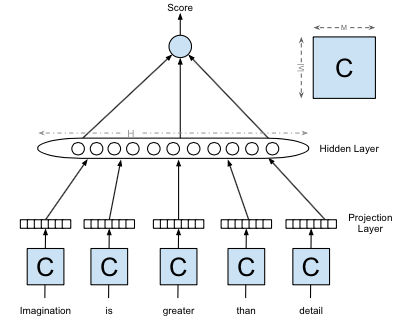1. Each of the words is transformed from a one-hot to a distributed representation via the application of a shared matrix $C$, and these representations are concatenated;
2. The hyperbolic tan of an affine transformation of this concatenation is calculated component-wise, yielding a “hidden” vector.
3. The components of this vector are combined via an affine transformation to yield the score.

So this neural network has three layers and the parameters are the shared matrix $C$ together with the two affine transformations.

The word embedding is given by the rows of the shared matrix $C$.

The models are trained using Theano for extensive periods of time (the authors mention “weeks”). The window size is taken to be radial length 2, the word embedding rank is 64 and the hidden layer size is 32.

To demonstrate the utility of the word representations, the authors the representations as initialisation for a model performing parts of speech tagging.

The paper was published at about the same time as word2vec (it does not refer to word2vec at all). The approach, the notation and the terminology, however, demonstrate that certain things that I had thought particular to word2vec were in fact already accepted practice, including:

• the use of discriminative tasks for training word embeddings
• sampling contexts by scanning a short window over text
• the use of the middle word in a context for the discriminative task
• dividing through by the “fan out” for initialisation (page 187, TBC)
• the symbols <S> and </S> for delimiting sentences

### A Unified Model for Word Sense Representation and Disambiguation

Chen, Liu, Sun, published in the conference proceedings of EMNLP 2014 (PDF).

The authors leverage the word2vec skipgram model and WordNet glosses (i.e. word sense definitions) for word sense disambiguation. This is achieved as follows:

1. A skipgram model is trained.
2. For each sense of a word according to WordNet, a vector is derived by taking the average of the content words in the WordNet definition (“gloss”) of that sense (“gloss vectors”)
3. The gloss vectors are used to identify the sense of a word occurrence by considering its dot product with the context of that occurrence. The sense whose gloss vector has the highest dot product with the context vector is chosen, as long as it is wins by a sufficient margin.

The authors are then able to train word sense vectors (distinct from the gloss vectors) by modifying the skip-gram objective. These word sense vectors are then used for similarity tasks and not for word sense disambiguation. It seems to me that it would have been simpler to annotate word occurrences in the corpus with the senses than to modify the objective.

Evaluation is performed for coarse-grained WSD (i.e. disambiguating homographs).

Independence assumptions in iterative word sense disambiguation
The authors disambiguate the senses of a words one word at a time, based upon the disambiguation that has already taken place. Two different strategies are considered for choosing the order in which to disambiguate the words in a context. These strategic approaches make a problematic independence assumption – that the sense of the word to be disambiguated is independent of the senses of the words not yet disambiguated. I haven’t read many WSD papers – I suspect these independence assumptions aren’t particular to the approach of the authors.

### GloVe: Global Vectors for Word Representations

Pennington, Socher, Manning, 2014.
PDF

GloVe trains word embeddings by performing a weighted factorisation of the log of the word co-occurrence matrix. The model scales to very large corpora (Common Crawl 840B tokens) and performs well on word analogy tasks.

Model
The cost function is given by:

$\displaystyle \sum_{i, j = 1}^V f(X_{i,j}) (u_i^T v_j + b_i + c_j – \text{log} X_{i,j})^2$

where:

• $V$ is the size of the vocabulary,
• $X$ denotes the word co-occurrence matrix (so $X_{i,j}$ is the number of times that word $j$ occurs in the context of word $i$)
• the weighting $f$ is given by $f(x) = (x / x_{\text{max}})^\alpha$ if $x < x_{\text{max}}$ and $1$ otherwise,
• $x_{\text{max}} = 100$ and $\alpha = 0.75$ (determined empirically),
• $u_i, v_j$ are the two layers of word vectors,
• $b_i, c_j$ are bias terms.

Note that the product is only over pairs $i, j$ for which $X_{i,j}$ is non-zero. This means that GloVe (in contrast to word2vec with negative sampling) trains only “positive samples” and also that we don’t have to worry about the logarithm of zero.

This is essentially just weighted matrix factorisation with bias terms:

Note that in the implementation (see below), the $X_{i,j}$ are not raw co-occurrence counts, but rather the accumulated inverse distance between the two words, i.e.

$\displaystyle X_{w, w’} := \sum_{\text{windows containing\ } w, w’} (\text{distance between\ } w, w’)^{-1}.$

I am fairly sure that the implementation of Adagrad is incorrect. See my post to the forum.

The factor weighting f

The authors go to some trouble to motivate the definition of this cost function (section 3).  The authors note that many different functions could be used in place of their particular choice of $f$, and further that their $\alpha$ coincides with that used by word2vec for negative sampling. I can’t see the relevance of the latter, however (in word2vec, the $0.75$th power it is used to define the noise distribution; moreover powering a value in the range $[0, 1]$ has a very different effect to powering a value in the range $[0, 100]$).

Graphing the function (see above) hints that it might have been specified more simply, since the non-linear region is in fact almost linear.

Word vectors
The resulting word embeddings ($u_i$ and $v_j$) are unified via a direct sum of their vector spaces.

The cosine similarity is used to find the missing word in word similarity tasks. It is not stated if the word vectors were normalised before forming the arithmetic combination of word vectors.

Source code
The authors take the exemplary step of making the source code available.

Evaluation and comparison with word2vec
The authors do a good job of demonstrating their approach, but do a scandalously bad job of comparing their approach to word2vec. This seems to reflect a profound misunderstanding on the part of the authors as to how word2vec works. While it has to be admitted that the word2vec papers were not well written, it is apparent that the authors made very little effort at all.

The greatest injustice is the comparison of the performance of GloVe with an increasing number of iterations to word2vec with an increasing number of negative samples:

The most important remaining variable to control
for is training time. For GloVe, the relevant
parameter is the number of training iterations.
For word2vec, the obvious choice would be the
number of training epochs. Unfortunately, the
code is currently designed for only a single epoch:
it specifies a learning schedule specific to a single
pass through the data, making a modification for
multiple passes a non-trivial task. Another choice
is to vary the number of negative samples. Adding
negative samples effectively increases the number
of training words seen by the model, so in some
ways it is analogous to extra epochs.

Firstly, it is simply impossible that it didn’t occur to the authors to simulate extra iterations through the training corpus for word2vec by simply concatenating the training corpus with itself multiple times. Moreover, the authors themselves are capable programmers (as demonstrated by their own implementation). The modification to word2vec that they avoided is the work of ten minutes.

Secondly, the notion that increasing the exposure of word2vec to noise is comparable to increasing the exposure of GloVe to training data is ridiculous. The authors clearly didn’t take the time to understand the model they were at pains to criticise.

While some objections were raised about the evaluation performed in this article and subsequent revisions have been made, the GloVe iterations vs word2vec negative sample counts evaluation persists in the current version of the paper.

Another problem with the evaluation is that the GloVe word vectors formed as the direct sum of the word vectors resulting from each matrix factor. The authors do not do word2vec the favour of also direct summing the word vectors from the first and second layers.

### A Graph-Based Method for Combining Collaborative and Content-Based Filtering

Phuong, Thang and Phuong (all from the Posts and Telecommunications Institute of Technology, Vietnam), 2008.

PDF obtainable here.

The authors present a recommendation system that incorporates user ratings of items (they work in the explicit rating context) and item-feature relations. The approach is graphical. Effectively, two weighted graphs with non-negative weights are constructed, network propagation is performed on both independently and the two resulting scorings are combined in a weighted sum. The first graph is directed represents user-item preference via the item features (where the user-feature preferences are computed heuristically from the given ratings and item-feature associations); thus in this graph all paths from user to item have length 2. The second graph is undirected and represents the purely positive user ratings of items and excludes the item features. The two graphs capture the content- and collaborative- aspects of the recommendation problem, respectively.

I find the approach lacks unity and is too heuristic. The unity suggested by the user/item/feature graph of Figure 1 is merely pictorial, since network propagation is actually performed on the two graphs described above (which are derived from this unified graph) separately. The two separate graphs are constructed heuristically, and this removes any claim the approach might have had to necessity.

The more obvious, unified, approach (network propagation on the user/item/feature graph) is unavailable here since the user-item associations may be negative. This would not be the case if the feedback were implicit (e.g. purchases). For this reason I will be interested to read the paper of Huang et al. cited by the authors – perhaps they use just such an approach. Furthermore, Huang et al. experiment with different network propagation algorithms (in this paper, a modification of an algorithm by Weston et al. is used).

The MovieLens dataset is used in the evaluations, which demonstrate the superiority of the authors approach over a purely content, a purely collaborative and a simple hybrid approach that merges the result sets of collaborative filtering and content recommendation computed separately.

Paper is clearly written.

### HybridRank: A Hybrid Content-Based Approach to Mobile Game Recommendations

Chow, Foo and Manai (all at Group Digital Life, Singtel, Singapore) 2014.

PDF.

The authors consider a personalised PageRank on a graph whose vertices represent items for recommendation and whose edge weights are determined by feature overlap (where features are e.g. category, tag). In order to incorporate collaborative information into the computation, they define the “teleport vector” (or “reset vector”) for a user to be the sum over all items they’ve interacted with of the corresponding rows of the behavioural item x item matrix (they call these “user correlation matrices”, somewhat confusingly).

This is a nice advance from “ItemRank” for incorporating item meta information into the recommendation process. In contrast to ItemRank, the authors work in the context of implicit feedback data. However, I think that the approach could be made more elegant by considering the users and tags (for example) as additional vertices in the graph – the reset vector would then just be the one-hot vector singling out the vertex corresponding to the user receiving the recommendations.

The authors carry out an enormous user survey and an impressive live production test to demonstrate the performance of their approach.

### ItemRank: A Random-Walk Based Scoring Algorithm for Recommender Engines

Marco Gori and Augusto Pucci, 2007 (from the IJCAI conference proceedings).

PDF.

The authors consider an application of PageRank to recommendation in the case where explicit ratings are available. The vertices of the graph represent items to be recommended, and the weight of the edge between any two vertices is proportional to the number of users that have interacted with both the corresponding items (thus the explicit ratings are not incorporated into the graph itself). To obtain recommendations for a particular user, the “reset-” (or “teleport-“) vector of is set to be the explicit ratings given by the user (0 is used for the absence of a rating), PageRank is run and then resulting importances are used to rank the items.

It seems to me that this set-up would be more sensible in contexts where the behavioural data was implicit (e.g. user looked at particular item) rather that explicit (user gave a particular rating to a particular item) – in the explicit context the use of the value 0 for the absence of rating can not be motivated.

The authors test their approach on the MovieLens dataset.

As the authors themselves note, PageRank had been used for personalised (more generally, deliberately biased) recommendation before their work (e.g. Haveliwala “Topic sensitive Pagerank”, 2002). The novelty here lies in the construction of the graph from the user-item interaction matrix.

### Language Understanding for Text-based Games using Deep Reinforcement Learning

Appeared on the arXiv, June 2015.

The joint work of Karthik Narasimhan, Tejas Kulkarni and Regina Barzilay.

The aim of the paper is to create an autonomous agent that solves quests in text-based adventure games. The agent has no knowledge of the underlying game state, and must decide upon what action to take based only upon the representation of the game state that is afforded by the game. In this sense it seeks to solve a similar problem to that of the now famous Atari deep learning paper. This is also an interesting model for how humans communicate with one another.

There are similarities in approach, moreover, in that both employ reinforcement learning. In contrast, this paper employs a Long-Short Term Memory network.

They use Evennia, a Python framework for building multiplayer online text games (used here in a single player context).

Adriaan S.: Q-learning does not scale well. (This could account for the small vocabulary used.)

### Perpendicularity and dimension

We show below that vectors drawn uniformly at random from the unit sphere are more likely to be orthogonal in higher dimensions.

In information retrieval and other areas besides, it is common to use the dot product of normalised vectors as a measure of their similarity. It can be problematic that the similarity measure depends upon the rank of the representation, as it does here — it means, for example, that similarity thresholds (for relevance in a particular situation) need to be re-calibrated if the underlying vectorisation model is retrained e.g. in a higher dimension.

Question
Does anyone have a solution to this? Is there a (of course, dimension dependent) transformation that can be applied to the dot product values to arrive at some dimension independent measure of similarity?

I asked this question on “Cross Validated”. Unfortunately, it has attracted so little interest that it has earned me the “tumbleweed” badge!

For those interested in the distribution of the dot products, and not just the expectation and the variance derived below, see this answer by whuber on Cross Validated.

### Marginal and Conditional Distributions of the Multivariate Gaussian

This is the standard, elementary arithmetic proof that the marginal and conditional distributions of the multivariate Gaussian are again Gaussian with parameters expressible in terms of the covariance matrix of the original Gaussian. We use the block multiplication of matrices.

I was surprised by how much work is required to show this, and feel moreover that the proof (while correct) fails to offer any intuitive understanding. Is there not a higher-level, co-ordinate free proof of this important result, perhaps one that uses characteristic properties of multivariate Gaussians?

Update: I received an answer to this question on Cross Validated from whuber. He uses a generative definition: the multivariate Gaussians are precisely the affine transformations of tuples of standard (mean zero, unit-variance) one-dimensional Gaussians. Using this definition, it follows quickly that the conditional and marginal distributions must be multivariate Gaussian.

### Word2vec weight initialisation

The initialisation of the weights in word2vec is not what I expected.

• syn1 The weights connecting the hidden- to the output-layer are initialised to zero (in both the hierarchical softmax and the negative sampling cases)
• syn0 The initial values for the weights connecting the input- to the hidden-layer are drawn uniformly and independently from the interval $[\frac{-1}{2n}, \frac{1}{2n} ]$, where $n$ is the rank of the hidden layer (i.e. number of hidden units.)

The range of interval from which the syn0 weights are sampled was chosen depends on the rank. I had presumed that this was to account for the dependency of the distribution of the dot product (and in particular the L2-norm) on the rank. However, estimating these distributions empirically, this doesn’t seem to be the case: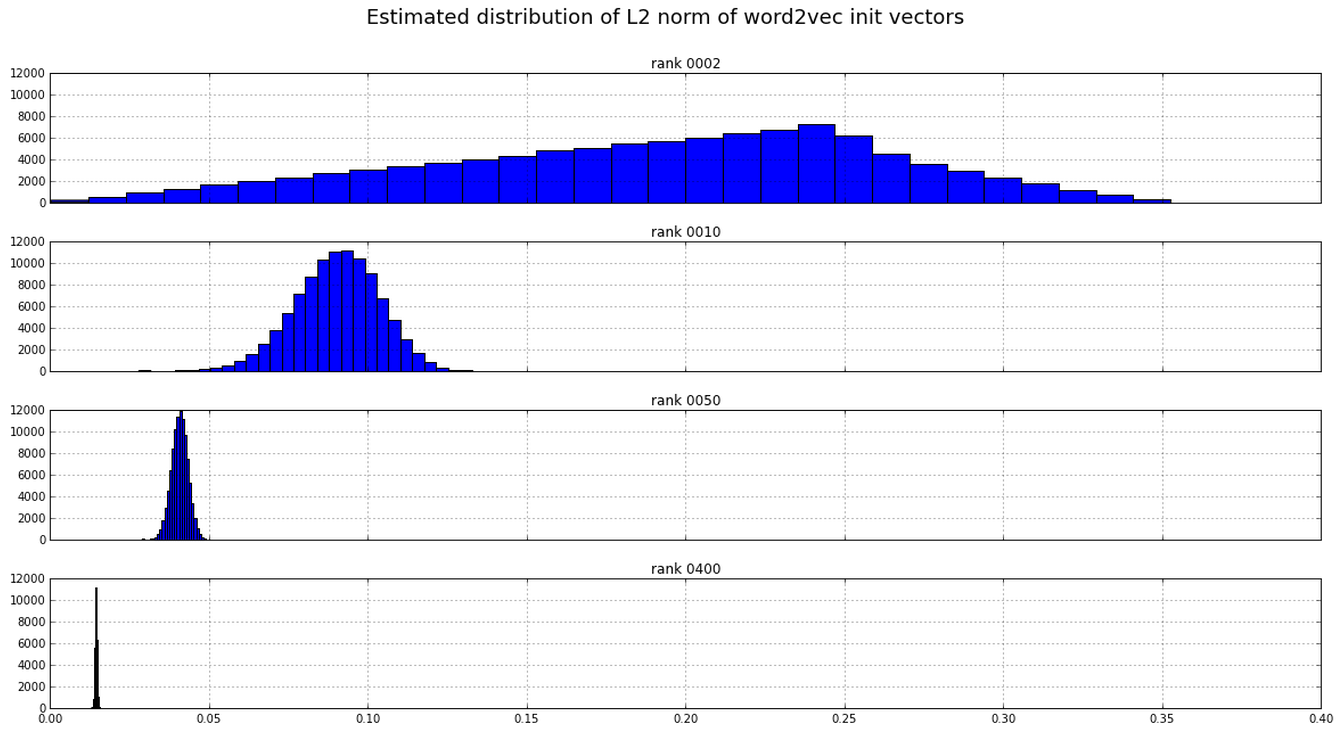According to Mikolov (in a helpful response in the word2vec google group), the initialisation of the weights was chosen empirically, since it seemed to work well.

Questions:

1. I was unable to derive an expression for the distribution of L2-norms mathematically. Can someone help with that?

### PageRank meets vectorial representations – "Ranking on Data Manifolds"

I came across this paper when following up on ideas I had when reading about TextRank for summarising documents. It is short, well written and very interesting, and was authored by Zhou, Weston, Gretton, Bousquet and Schölkopf (all then at the Max Planck Institute for Biological Cybernetics, Tübingen) in 2004. (PDF).

The authors consider the problem of ranking objects by relevancy to one or more query objects in the case where the objects have a vectorial representation. This is done using the PageRank algorithm on a graph in which the vertices represent the objects and the edges weights are computed using e.g. an RBF kernel (or normalised dot-product, if the vectors are non-negative).

One advantage of this approach is that it generalises naturally to multiple query vectors. The query vectors are simply treated as a complete list of possible (re)starting points for the PageRank random walk. This contrasts with the typical PageRank case, where all pages are possible starting points. Note that this list of starting points need not be binary — it can rather be a probability distribution over the objects representing user preference.

The PageRank approach is shown to perform much better than Euclidean nearest neighbours search on real world data sets (MNIST digits and newsgroup posts are considered). In both cases, the datasets have labels. The query data points are chosen from a single class, and the ranking problem is treated as one of binary classification, i.e. finding the distinguishing the objects of the same class from those of all other classes. The ranking is used to calculate a ROC curve, and the area under this curve is used as a performance measure.

The evaluation considers the case of multiple query vectors, as well. The Euclidean nearest neighbours, in the multiple query vector case, are aggregated by taking the minimal distance to a query vector (i.e. “disjunctively”).

The PageRank method is visually contrasted with the Euclidean nearest neighbours case using the MNIST data set. Below are the 99 best ranked results in PageRank case (left) and the Euclidean case (right) (99 = 10 x 10 – 1 query). Not only does the left panel contain no threes, but the twos are more homogeneous.

The graph that the authors construct is not complete. Rather, edges are added, beginning with those most heavily weighted (but excluding self-loops) until the graph is connected. For example, in the case of the two sickle moons:

Hyperparameters
An RBF kernel function is used in some cases to define the edge weighting between nodes (see step 2 of the algorithm). Note that the variance $\sigma$ of the RBF kernel needs to be fitted using cross-validation. This is no problem for labelled datasets like MNIST, but would be problematic for e.g. the sickle moons data.

It’s not a random walk
Note that, due to the “symmetric normalisation” that is applied to the affinity matrix $W$ (see step 3 of the algorithm), this is not a random walk — the columns of the normalisation $S$ do not sum to 1, so the iterative procedure of step 4 will not map probability distributions to probability distributions. Given that we only want to rank the nodes, not derive a probability distribution over them, this is not necessarily a problem.

Why symmetric normalisation? Dengyong was kind to explain the motivation for this (via correspondence). This normalisation was used in order to avoid over-emphasising points in high density regions, and because it is the normalised graph Laplacian (I haven’t looked into this). Dengyong added that these reasons were not strong, and that other alternatives should be investigated.

Corrections to the paper
There are some mistakes in the paper. In particular, there is a problem with Theorem 2, pointed out by begab on reddit when I shared this post. Begab points out that $DU \neq DU$. The problem is larger than this, in fact, since the claim that the steady state of a PageRank random walk on a connected, undirected graph does not hold. There is the following counterexample, for instance.

Questions

1. Who has taken this research further?
2. In both of the cases considered, the vector representations of the objects are rather poor (pixel on/off and tf-idf). How much better is this approach if dimension reduction is first applied to the vectors?

### Block Multiplication of Matrices

(We needed this to derive the conditional distribution of a multivariate Gaussian).

Consider a matrix product $AB$. Partition the two outer dimensions (i.e. the rows of $A$ and the columns of $B$) and the one inner dimension (i.e. the columns of $A$ and the rows of $B$) arbitrarily. This defines a “block decomposition” of the product $AB$ and of the factors $A, B$ such that the blocks of $AB$ are related to the blocks of $A$ and $B$ via the familiar formula for components of the product, i.e.

$(AB)_{m,n} = \sum_s A_{m,s} B_{s,n}$.

Pictorially, we have the following:

Arithmetically, this is easy to prove by considering the formula above for the components of the product. The partitioning of the outer dimensions comes for free, while the partitioning of the inner dimension just corresponds to partitioning the summation:

$(AB)_{m,n} = \sum_s A_{m,s} B_{s,n} = \sum_i \sum_{s_i \leq s \leq s_{i+1}} A_{m,s} B_{s,n}$.

Zooming out to a categorical level, we can see that there is nothing peculiar about this situation. If, in an additive category, we have three objects $X, Y, Z$ with biproduct decompositions, and a chain of morphisms:

$X \xrightarrow{\varphi_B} Y \xrightarrow{\varphi_A} Z$

then this “block decomposition of matrices” finds expression as a formula in $\text{End}(X, Z)$ using the injection and projection morphisms associated with each biproduct factor.

### TextRank: Bringing Order into Texts

Published in 2004 (PDF) by Rada Mihalcea and Paul Tarau.

I picked this paper up after seeing that it had been integrated into GenSim (see also this article by the contributor to gensim and others). The authors (of the original paper) apply the PageRank algorithm to graphs constructed from text for the purposes of keyword extraction and summarisation. These two approaches they name (somewhat unnecessarily, I feel) TextRank.

You can test how it works yourself e.g. the Python implementation here.

In the case of keyword extraction, the graph has words as vertices (in the best case, only nouns and adjectives) and the (undirected) edges represent co-occurrence within a fixed length window.

In the case of summarisation, the graph has sentences as vertices and the graph is complete. The weight of each edge can be determined by any sentence similarity function. The authors consider the case where sentence similarity is measured by word overlap, normalised by sentence length. If a vectorial representation of the sentences is available, then e.g. the cosine similarity could be used instead. The authors extend the definition of PageRank to deal with weighted graphs.

The advantage of the TextRank approaches is that nothing needs to be learnt — there is no machine learning involved at all. The keyword extraction and summarisation make relatively loose assumptions about the language of the text and apply equally well to documents from unseen domains. TextRank is, however, entirely heuristic. The theory leaves off where the authors begin (that is, with PageRank). The authors do present an interesting application of PageRank, however.

Questions:

• Has anyone tried the summarisation out using a vectorial representation of sentences and the cosine similarity? Other than bag-of-words?
• If we use e.g. the RBF kernel $e^{- \| x_1 – x_2\|}$ for the edge weight between two vectors $x_1, x_2$, what points does PageRank tend to choose from a (multimodal) data sample? Related is perhaps this article. (See also my summary).

### Document Classification by Inversion of Distributed Language Representations

This is a note on the arxiv by Matt Taddy from April 2015. It reads very clearly and has a simple point to make: language modelling techniques can be used in classification tasks by training a separate language model for each class; documents are assigned to the class of the model where the document has the highest likelihood (hence “inversion”). In our discussion, we assume a uniform prior over the classes.

Taddy considers the particular case of predicting the sentiment of Yelp reviews at different levels of granularity. Different approaches are considered:

• word2vec inversion is inversion in the sense described above where document vectors are taken as the average of the word vectors of the constituent words;
• phrase regression, where separate logistic regression models are trained for each output class, taking as input phrase count vectors;
• doc2vec regression, is as per phrase regression, but taking as input one of:
• doc2vec DBOW
• doc2vec DM
• doc2vec DBOW and DM combined, i.e. in direct sum
• MNIR, the authors own Multinomial Inverse Regression

Three separate classification tasks are considered, labelled “a”, “b” and “c” in the diagram below, representing two-, three- and five-class sentiment classification.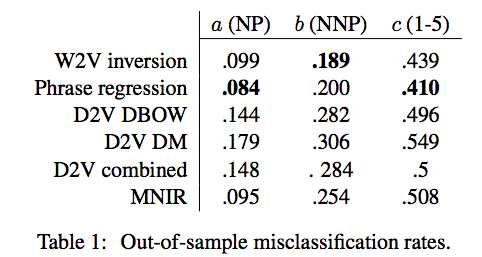As illustrated in the following figure, only the word2vec inversion technique would do a decent job when the gravity of a misclassification is considered (so penalising less if, e.g. predicted star rating is off by only one star):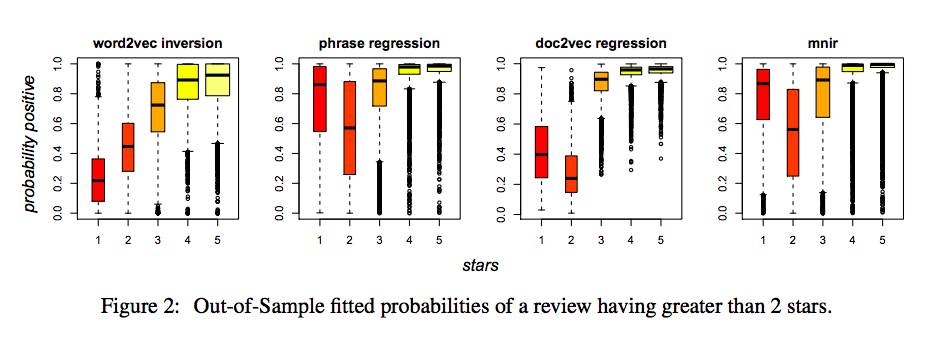Missing from Taddy’s comparison is inversion using the document vectors, though this is certainly the sort of thing his paper suggests might work well. Also missing is regression using the document vectors obtained as aggregates of word vectors.

### Document Embedding with Paragraph Vectors

Presented at NIPS 2014 (PDF) by Dai, Olah, Le and Corrado.

## Model

The authors consider a modified version of the PV-DBOW paragraph vector model. In previous work, PV-DBOW had distinguished words appearing in the context window from non-appearing words given only the paragraph vector as input. In this modified version, the word vectors and the paragraph vectors take turns playing the role of the input, and word vectors and paragraph vectors are trained together. That is, a gradient update is performed for the paragraph vector in the manner of regular PV-DBOW, then a gradient update is made to the word vectors in the manner of Skipgram, and so on. This is unfortunately less than clear from the paper. The authors were good enough to confirm this via correspondence, however (thanks to Adriaan Schakel for communicating this). For the purposes of the paper, this is the paragraph vector model.

The representations obtained from paragraph vector (using cosine similarity) are compared to those obtained using:

• an average of word embeddings
• LDA, using Hellinger distance (which is proportional to the L2 distance between the component-wise square roots)
• paragraph vector with static, pre-trained word vectors

In the case of the average of word embeddings, the word vectors were not normalised prior to taking the average (confirmed by correspondence).

## Corpora

Two corpora are considered, the arXiv and Wikipedia:

• 4.5M articles from Wikipedia, with a vocabulary of size 915k
• 886k articles from the arXiv, full texts extracted from the PDFs, with a vocabulary of 970k words.

Only unigrams are used. The authors observed that bigrams did not improve the quality of the paragraph vectors. (p3)

## Quantitative Evaluation

Performance was measured against collections of triples, where each triple consisted of a test article, an article relevant to the test article, and an article less relevant to the test article. While not explicitly stated, it is reasonable to assume that the accuracy is then taken to be the rate at which similarity according to the model coincides with relevance, i.e. the rate at which the model says that the relevant article is more similar than the less relevant article to the test article. Different sets of triples were considered, the graph below shows performance of the different methods relative to a set of 172 Wikipedia triples that the authors built by hand (these remain unreleased at the time of writing).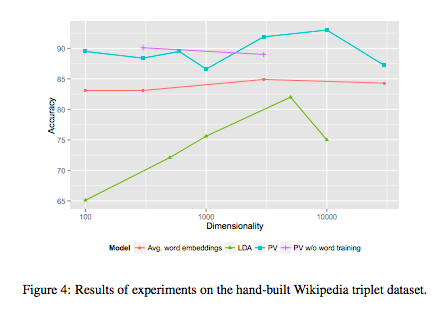It is curious that, with the exception of the averaged word embeddings, the accuracy does not seem to saturate as the dimension increases for any of the methods. However, as each data point is the accuracy of a single training (confirmed by correspondence), this is likely nothing more than the variability inherent to each method. It might suggest, for example, that the paragraph vectors method has a tendency to get stuck in local minima. This instability in paragraph vector is not apparent, however, when tested on the triples that are automatically generated from Wikipedia (Figure 5). In this latter case, there are many more triples.

Performance on the arXiv is even more curious: accuracy decreases markedly as the dimension increases!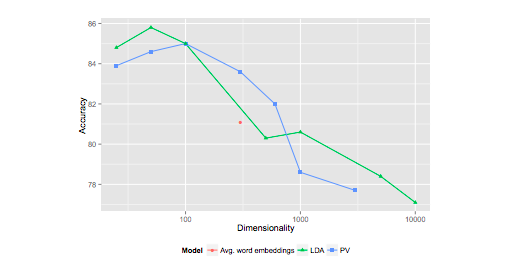## Implementations

I am not sure there are any publicly available implementations of this modified paragraph vectors method. According to Dai, the implementation of the authors uses Google proprietary code and is unlikely to be released. However it should be simple to modify the word2vec code to train the paragraph vectors, though some extra code will need to be written to infer paragraph vectors after training has finished.

I believe that the gensim implementation provides only the unmodified version of PV-DBOW, not the one considered in this paper.

It is interesting that the paragraph vector is chosen so as to best predict the constituent words, i.e. it is inferred. This is a much better approach from the point of view of word sense disambiguation than obtaining the paragraph vector as a linear image of an average of the word vectors (NMF vs PCA, in their dimension reductions on bag of words, is another example of this difference).

Questions

1. Is there is an implementation available in GenSim? (see e.g. this tutorial).
2. (Tangent) What is the motivation (probabilistic meaning) for the Hellinger distance?

### Expectation-Maximisation and Gaussian Mixture Models

Below are notes from a talk on Expectation Maximisation I gave at our ML-learning group. Gaussian mixture models are considered as an example application.

The exposition follows Bishop section 2.6 and Andrew Ng’s CS229 lecture notes. If you weren’t at the seminar, then it is probably better to read one of these instead.

Another useful reference is likely the 1977 paper by Dempster et al. that made the technique famous (this is something I would have liked to have read, but didn’t).

Questions

1. I still don’t understand how EM manages to (reportedly) work so well, given that the maximisation chooses for the next parameter vector precisely the one that reinforces the “fantasy” completions of the data made by the previous parameter vector. I would not have considered it a good learning strategy. It contrasts greatly with, for example, the learning strategy of a restricted Boltzmann machine, in which, at each iteration, the parameters are adjusted so as to correct the model’s fantasy towards producing the observed data.
2. Can we offer a better argument for why maximisation of the likelihood for latent variable models is difficult?
3. Is the likelihood of an exponential family distribution convex in the parameters? This is certainly the case for e.g. the mean of a Gaussian. Does this explain why the maximisation of the constructed lower bound for the likelihood is easy?

### Support Vector Machine Active Learning with Applications to Text Classification

This is an old but interesting paper from 2001 by Simon Tong (now at Google) and Daphne Koller (who launched Coursera with Andrew Ng), both then at Stanford. (PDF here)

As the title suggests, the authors apply SVMs to text classification using active learning techniques.  This is the first active learning paper I’ve read, and it has provided me with some useful notions.  I’ll be reading further in this field, as building e.g. a Tinder-like application for finding research articles of interest or for “e-Discovery” applications in litigation interest me.  These are both examples of relevance feedback for transduction since the classifier will be applied to a collection of (unlabelled) samples that we know of in advance (in contrast, applying to unseen samples, e.g. for e-mail filtering, is induction).  In machine learning, recognition of this distinction is due to Vapnik.

The authors introduce the notion of a version space for a SVM trained on linearly separable data: it is the set of all hyperplanes that separate the two classes in the training data, thought of as points on the unit sphere by taking the unit normal to the hyperplane.  The area of the version space is thus a measure of our uncertainty of the true decision boundary.  The authors propose an active learning approach that successively “queries” (i.e. requests the label for) a sample that would maximally reduce the area of the version space.

Three heuristic methods are proposed for choosing a such a sample: SimpleMargin, MaxMin Margin and Ratio Margin.  The latter two outperform the former, but are significantly more expensive, computationally, since they require the training of SVMs and the cost of training a SVM is polynomial in the size of the training set.  All three active learning methods are much better than random.

Linear separability of the training examples is not a problem for the authors, since they operate of word count vectors (the vocabulary size = rank is about 10k).  In the case where a dense, lower dimensional vectorisation was used, linear separability would likely still hold, particularly if kernels were used.

The perform experiments using the Reuters corpus and a newsgroup collection.

Questions

• Active learning by successively reducing the area of the version space is an interesting approach. I wonder if, more generally, we might think of choosing a sample that maximally reduces the entropy of the posterior distribution?

### Entropy of the Normal Distribution

Here we calculate the entropy of the normal distribution and show that the normal distribution has maximal entropy amongst all distributions with a given finite variance.

The video belong concerns just the calculation of the entropy, not the maximality property.

### Shannon: averaging operations can only increase the entropy

Shannon makes this claim in his 1948 paper and lists this property as one of his justifications for the definition of entropy. We give a short proof of his claim.

### Matrix Factorisation and the Eigendecomposition of the Gram Matrix

Here we consider the problem of approximately factorising a matrix $X$ without constraints and show that solutions can be generated from the orthonormal eigenvectors of the Gram matrix $X^T X$ (i.e. of the sample covariance matrix).

For this we need the eigendecomposition of real symmetric matrices.

Questions, all related to one another:

• What other solutions are there?
• (Speculative) can we characterise the solutions as orbits of the orthogonal group on the solutions above, and on those solutions obtained from the above by adding rows of zeros to $B$?
• Under what constraints, if any, are the optimal solutions to matrix factorisation matrices with orthonormal rows/columns? To what extent does orthogonality come for free?

### Estimation Theory and the Bias-Variance Trade-Off

Below is a (rough!) draft of a talk I gave at the Berlin Machine-Learning Learning-Group on the bias-variance trade-off, including the basics of estimator theory, some examples and maximum likelihood estimation.

### Eigendecomposition of real, symmetric matrices

We show that a real, symmetric matrix has basis of real-valued orthonormal eigenvectors and that the corresponding eigenvalues are real.  We show moreover that these eigenvalues are all non-negative if and only if the matrix is positive semi-definite.

### Literary treasure hunting with the Lateral API

A good friend, Sam Matthews, recently gave a talk in December 2014 at a conference of the Australian Modernist Studies Network on “Transnational Modernisms”. Sam spoke about his discovery of a reference to a print-shop from Balzac’s “Two Poets” in Christina Stead‘s novel Seven Poor Men of Sydney. Sam later suggested that I check if we couldn’t use Lateral’s text matching service (the “Recommender (BYO documents!)” API) to confirm this reference to Balzac and potentially uncover other ones. As you’ll see below, the preliminary results are very encouraging. This is hardly a conclusive experiment, but

In case you would like to search for references to Balzac’s works yourself, you can do so by reusing the API key I created: b4de9b9183df4cbf8d70cde15609800a .

This is how I proceeded:

1. I downloaded the Complete works of Balzac from Project Gutenberg. This gives one HTML file for each of Balzac’s works.
2. I split each work into paragraphs, labelling the paragraphs by their work and position within the work. Balzac wrote many paragraphs, it turns out!
3. I subscribed to the API at Lateral, obtaining an API key.
4. I installed Francis Tzeng’s python package for accessing the Lateral API
5. Using the python package, I added the paragraphs of Balzac to the Lateral recommender. Short paragraphs containing not enough meaningful words were rejected; in total, the number of meaningful paragraphs of Balzac indexed was over 21,000.
6. Again using the python package, I searched for the closest paragraphs of Balzac to the passage of Stead that Sam had indicated to me (see below).

The passage of Stead’s novel that evokes the print-shop appears below (from Chapter 3):

devil’s kitchen where  the word is made bread … triangular park … A wide old doorway opened beside the tobacconist’s shop, and over it was a name, white on blue, “Tank Steam Press, Ground Floor.” The tobacconist owned the old single-storey building and rented out to several establishments the mouldy apartments of the ground and first floor. In the attic was the man who did heliogravure. The building had once been a private house. Its court was now a cart-dock and opened into the other street. Its first-floor bathroom at the head of the stairs contained the old water-closet, used by all the workers in the house, a gas-ring to make tea, and the usual broken chairs and out of-date telephone directories. The distinctive smell of the building came from this closet and from the printing-ink.Joseph walked through the old doorway, went by a staircase and entered the large airy double room occupied by the Press. He opened the glass back-door and moved about among the presses, curiously inspecting the jobs in their various stages, picking up a paper, looking through the bills on a bill-hook, putting his finger in the dust in the little glassed-in office of Chamberlain, the owner, and shutting off the stove, lighted by the cleaner, because the day was warm enough.

Below are the paragraphs of Balzac that are semantically closest to the text above, according to Lateral. As you can see, the 1st and the 9th closest paragraphs (of over 21,000!) indeed come from “Two Poets”, and inspection reveals that they indeed concern the printshop! You can click the links to fetch the corresponding paragraphs using the API. The intermediately ranked results seem to be architectural descriptions.

 [ { "distance": 0.034905, "document_id": "TWO POETS-00019" }, { "distance": 0.035945, "document_id": "THE COLLECTION OF ANTIQUITIES-00557" }, { "distance": 0.037409, "document_id": "SONS OF THE SOIL-01139" }, { "distance": 0.038067, "document_id": "A MAN OF BUSINESS-00034" }, { "distance": 0.038168, "document_id": "URSULA-01020" }, { "distance": 0.038216, "document_id": "COUSIN PONS-01938" }, { "distance": 0.03837, "document_id": "COLONEL CHABERT-00023" }, { "distance": 0.038545, "document_id": "COUSIN BETTY-01508" }, { "distance": 0.038823, "document_id": "TWO POETS-00018" }, { "distance": 0.038891, "document_id": "RISE AND FALL OF CESAR BIROTTEAU-01382" }, { "distance": 0.039151, "document_id": "THE RED INN and others-00045" }, { "distance": 0.039195, "document_id": "THE LESSER BOURGEOISIE(The Middle Classes)-00635" }, { "distance": 0.039369, "document_id": "SCENES FROM A COURTESAN'S LIFE-00761" }, { "distance": 0.039377, "document_id": "THE TWO BROTHERS-00663" }, { "distance": 0.039471, "document_id": "HONORINE-00036" }, { "distance": 0.039808, "document_id": "Z. MARCAS-00043" }, { "distance": 0.039896, "document_id": "RISE AND FALL OF CESAR BIROTTEAU-00623" }, { "distance": 0.040041, "document_id": "THE VILLAGE RECTOR-00313" }, { "distance": 0.040253, "document_id": "A WOMAN OF THIRTY-00700" }, { "distance": 0.04031, "document_id": "CATHERINE DE' MEDICI-01059" } ] 

## Lancichinetti et al 2015

http://arxiv.org/abs/1402.0422

(forwarded by Schakel)

LDA is a generative probabilistic topic model. The authors generate toy models using synthetic documents of words from distinct natural languages. This is in accordance with the generative model posited by LDA, where the topics here are the languages. They then calculate the likelihood of the desired (i.e. generating) solution and the likelihood of various deformed solutions, and show that in quite normal cases the generating solution can have a lower likelihood than a deformed solution.

They further show that the generating solution is often not obtained in practice by LDA and its standard methods of optimisation, even in the normal case where the generating solution is the unique global maximum of the likelihood function.

They describe an pragmatic (non-probabilisitic) approach to topic modelling, which involves first clustering words by detecting communities in the (denoised) word co-occurrence graph, and using these clusters to (somehow) choose initial values for PLSA or LDA to obtain a better solution.

They demonstrate how much better their method performs on their synthetic data.

I find the results of the authors vindicating. I have found the esteem of the machine learning community for LDA so at odds with my own experience of its performance that I wondered if I had misunderstood something. In the realm of information retrieval, we found LDA to be consistently out-performed by the non-probabilistic decompositions of PCA and NMF.

It is not too hard to find support for what I sense might be considered an unpopular opinion:

“Performance of LDA has never significantly surpassed PLSI (in fact we often found inferior results) which is the reason we left them out”

The authors of this paper undertook to investigate the short-comings of LDA by constructing some toy models. As they suggest themselves, it is not a new idea to create a toy model, but we don’t seem to do enough of it in machine learning.